# 什麼是資料結構？

• 資料結構是計算機儲存、組織資料的方式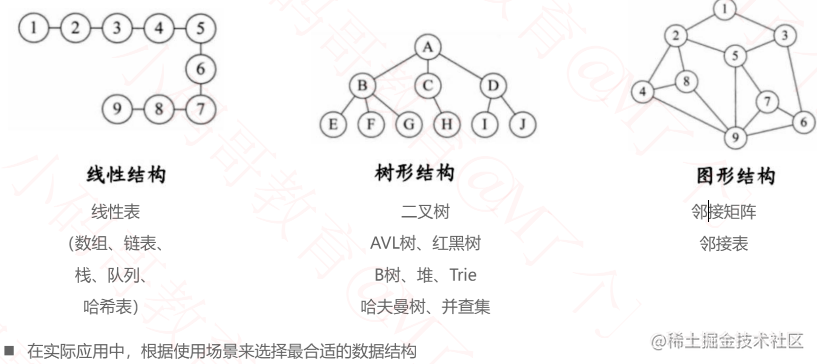# 一、線性結構, 線性表

• 線性表是具有n個相同型別元素的有限序列（n >= 0）
• 常見的線性表有: 陣列、連結串列、棧、佇列、雜湊表(散列表)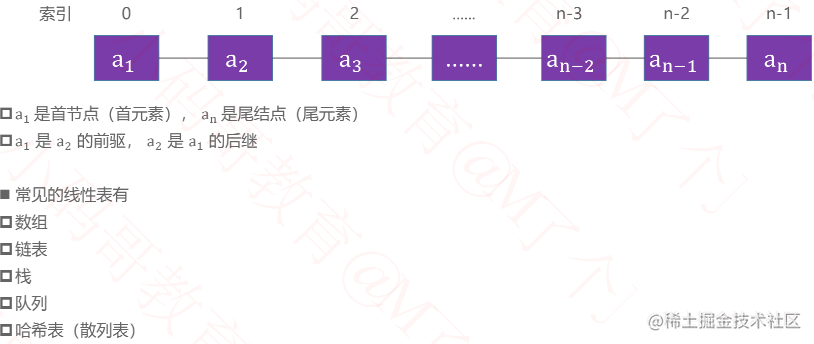## 基本概念

### 時間複雜度

• 大O表示法中, 時間複雜度的公式是: T(n) = O(f(n)), 其中f(n)表示每個程式碼執行次數之和, 而O表示正比例關係, 這個公式的全稱是:演算法的漸進時間複雜度.
• 常見的時間複雜度量級從上至下越來越大:
• 常數階O(1)
• 對數階O(logN)
• 線性對數階O(nlogN)
• 平方階O(n²)
• 立方階O(n³)
• K次方階O(n^k)
• 指數階O(2^n)

### 空間複雜度

• 空間複雜度是對一個演算法在執行過程中臨時佔用儲存空間大小的一個量度, 同樣反映的是一個趨勢, 我們用S(n)來定義.
• 空間複雜度比較常用的有: O(1)、O(n)、O(n²).
• 如果演算法執行所需要的臨時空間不隨著某個變數n的大小而變化, 即此演算法空間複雜度為一個常量, 可表示為O(1) ```// 程式碼中的i、j、m所分配的空間都不隨著處理資料量變化, 因此它的空間複雜度S(n) = O(1) int j = 1; int j = 2; ++i; j++; int m = i + j;```
• 空間複雜度O(n) ```int[] m = new int[n]; for (i = 1; i <= n; ++i) { j = i; j++ }```
• 這段程式碼中, 第一行new了一個數組出來, 這個資料佔用的大小為n, 這段程式碼的2-6航, 雖然有迴圈, 但沒有再分配新的空間, 因此, 這段程式碼的空間複雜度主要看第一行即可, 即S(n) = O(n);

## 1. 陣列（Array）

• 陣列是一種順序儲存的線性表， 所有元素的記憶體地址是連續的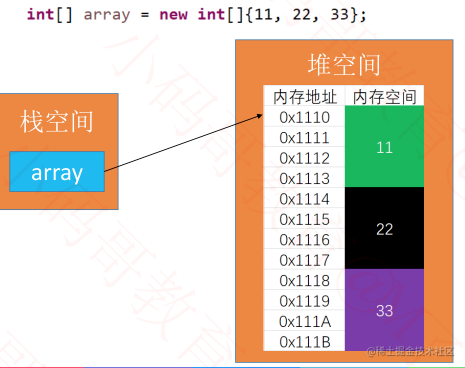• 很多程式語言中， 陣列都有個缺點，無法動態修改容量
• 實際開發中， 我們更希望陣列的容量是可以動態改變的

### 動態陣列的介面設計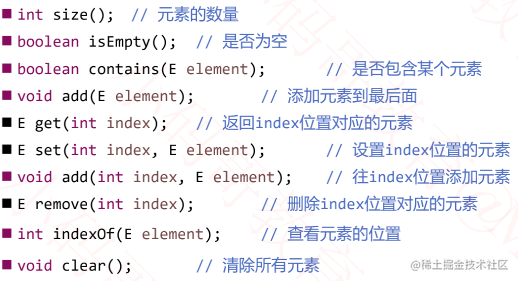#### 動態陣列的設計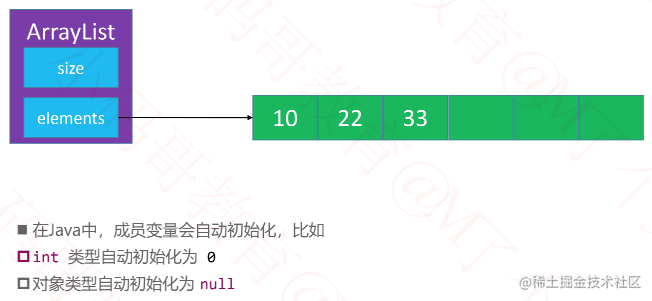- 新增元素 add(E element)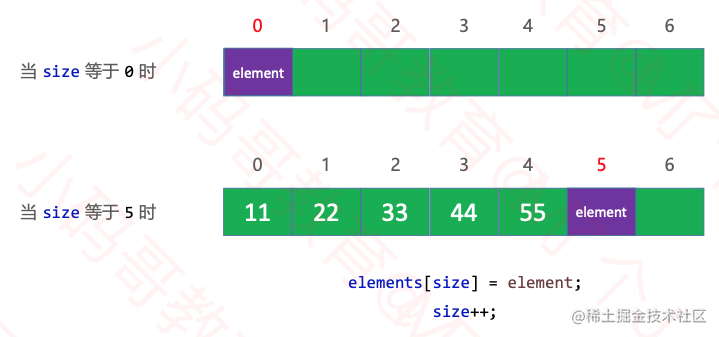• 列印陣列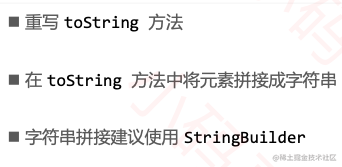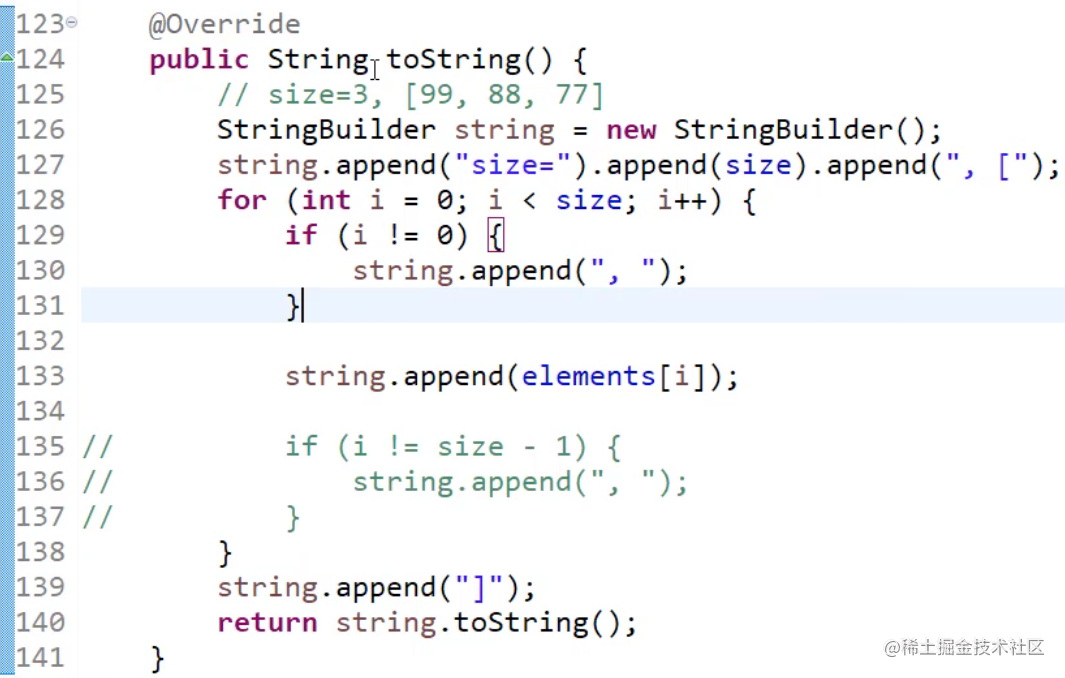• 刪除元素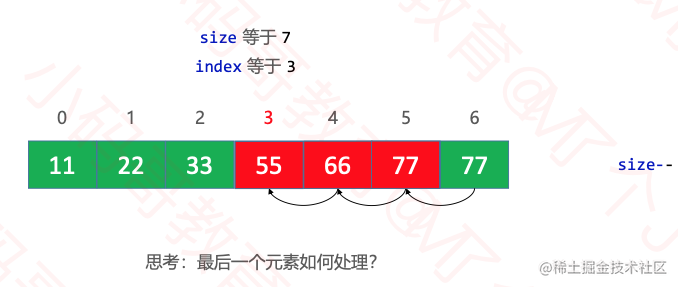- `new`申請的是連續的記憶體空間，不能中間挖掉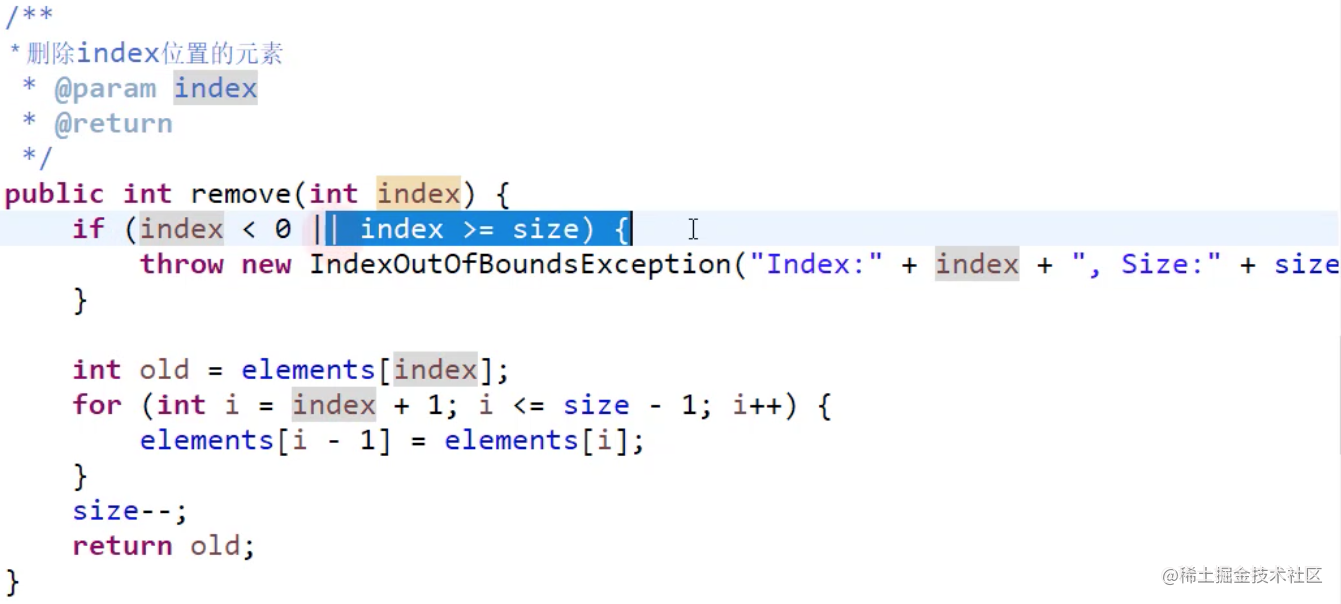• 插入新增元素 `add(int index, E element)`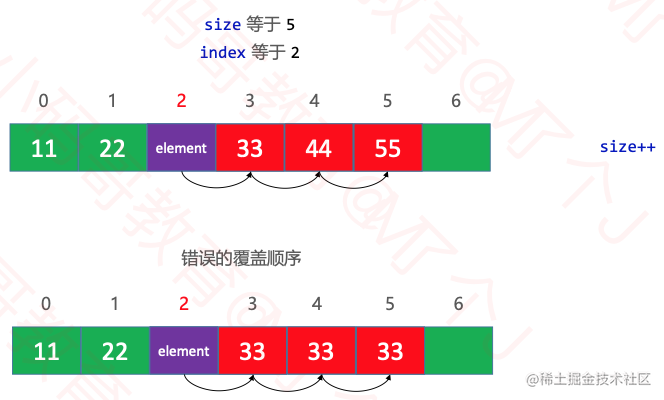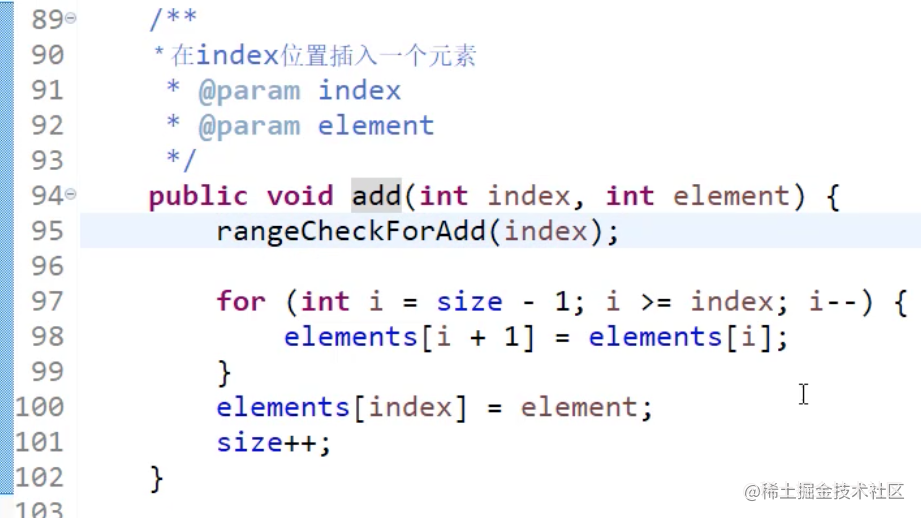- 把合規判斷抽取出來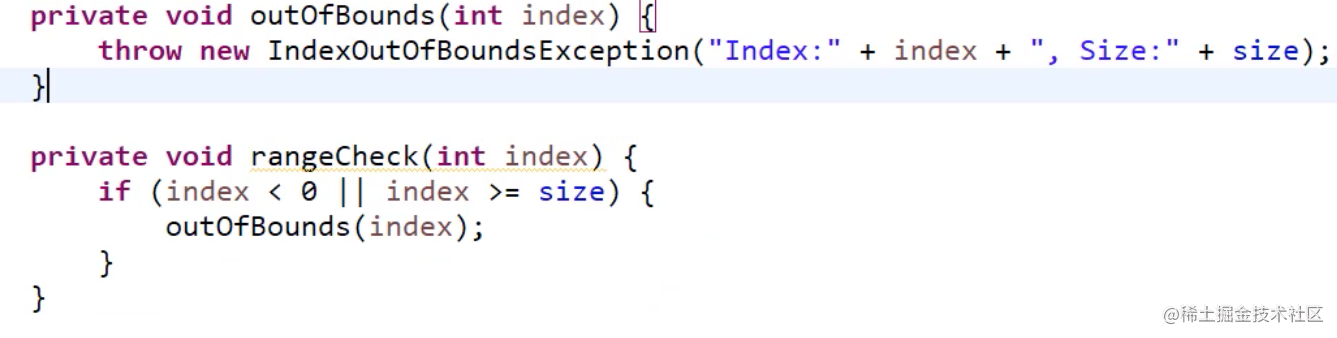• 動態陣列有個明顯的缺點
• 可能會造成記憶體空間的大量浪費
• 連結串列到多少記憶體就申請多少記憶體
• `連結串列`是一種`鏈式儲存`線性表, 所有元素的記憶體地址不一定是連續的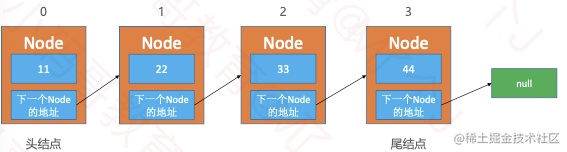### 反轉連結串列

• 輸入: 1->2->3->4->5->NULL
• 輸出: NULL->5->4->3->2->1

#### 遞迴法翻轉連結串列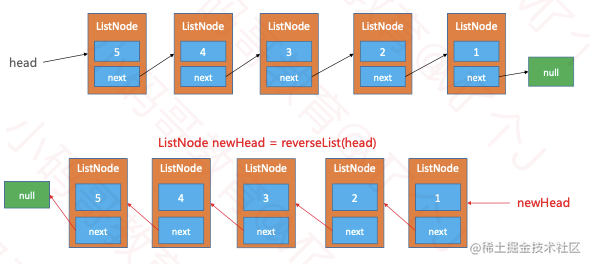• `ListNode newHead = reverseList(head.next)`做的事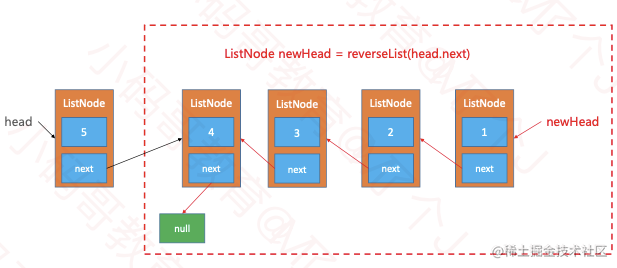``` // 遞迴的方式反轉連結串列 Java: public ListNode reverseList(ListNode head) { // 1. 傳入的引數合法性 || 遞迴的終止條件 if (head == null || head.next == null) return head;

}

Swift: /* * Definition for singly-linked list. * public class ListNode { * public var val: Int * public var next: ListNode? * public init() { self.val = 0; self.next = nil; } * public init( val: Int) { self.val = val; self.next = nil; } * public init( val: Int, _ next: ListNode?) { self.val = val; self.next = next; } * } / class Solution { func reverseList(_ head: ListNode?) -> ListNode? { // 採用遞迴的方式翻轉連結串列 // 1. 傳入的引數合法性 || 遞迴的終止條件 if head == nil || head?.next == nil { return head }

``````    // 2. 遞迴, 一直遞迴到連結串列的最後一個結點, 該結點就是反轉後的頭結點
// 3. 每次函式在返回過程中, 將當前結點的後一個結點的指標指向當前結點, 並斷開後一個結點指向後方的指標
// 4. 斷開當前結點指向後一個結點的指標, 並指向nil, 從而實現連結串列尾部開始的區域性反轉

// 5. 返回反轉後的連結串列, 當遞迴函式全部出棧後, 連結串列反轉完成.
}
``````

} ``` 反轉連結串列力扣連結

### 雙向迴圈連結串列

#### 雙向迴圈連結串列新增 - add(int index, E element)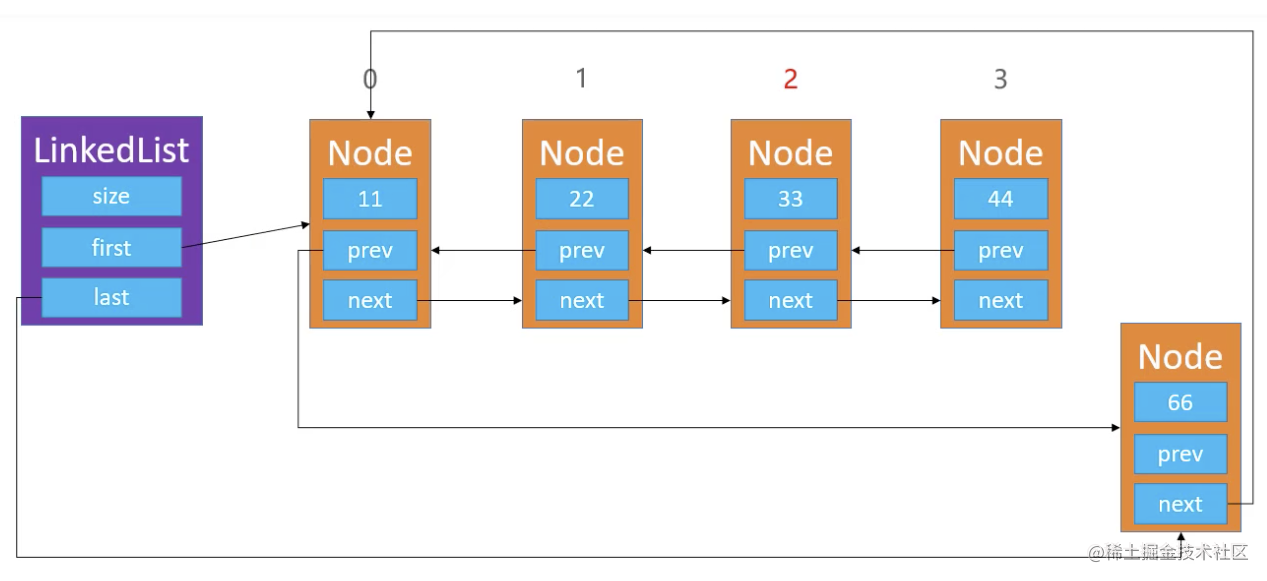#### 雙向迴圈連結串列刪除 - remove(int index, E element)

``` public E remove(int index) { rangeCheck(index);

``````Node<E> node = first;
if (size == 1) {
first = null;
last = null;
} else {
node = node(index);
Node<E> prev = node.prev;
Node<E> next = nodex.next;
prev.next = next;
next.prev = prev;

if (node == first) {// index == 0
first = next;
}
}

size--;
return node.element;
``````

} ```

### 約瑟夫問題(Jesephus Problem)

• 數到三開槍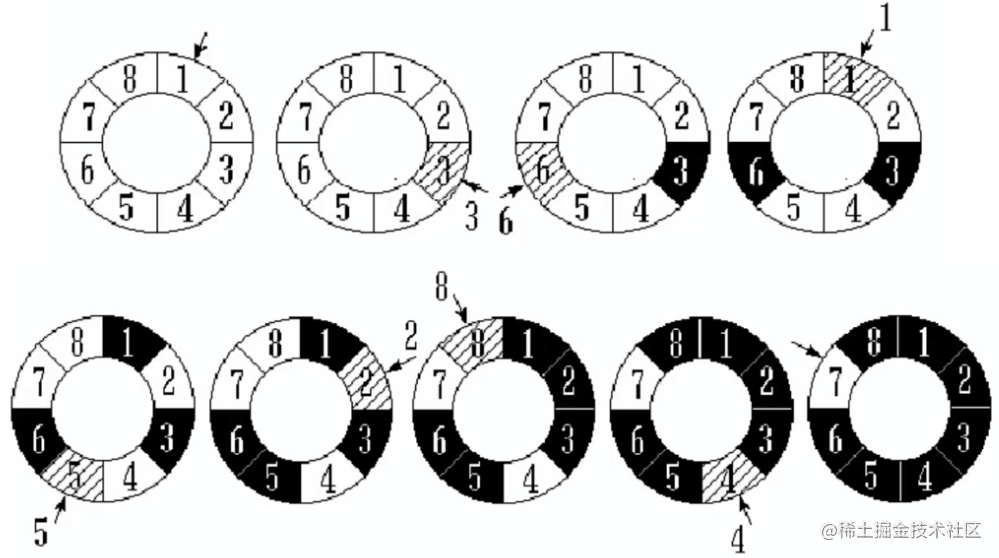• 可以考慮增設1個成員變數、3個方法
• current: 用於指向某個節點
• void reset(): 讓current指向頭結點`first`
• E next(): 讓`current`往後走一步, 也就是`current = current.next`
• E remove(): 刪除`current`指向的節點, 刪除成功後讓`current指向下一個節點`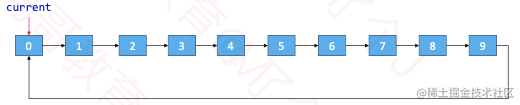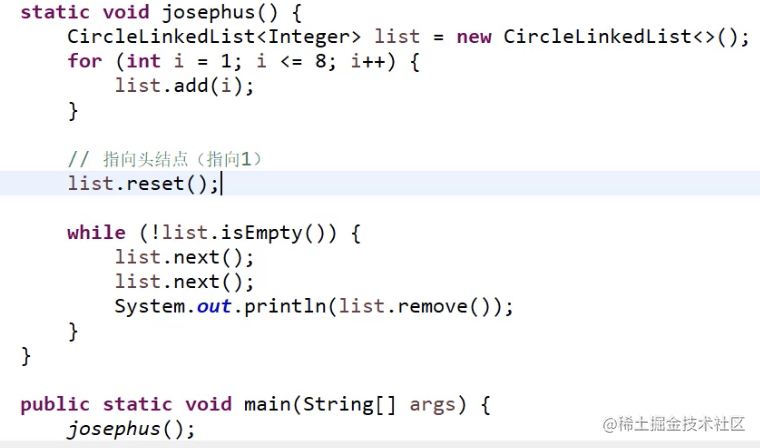### 靜態連結串列

• 數組裡面存放兩個元素, 模擬連結串列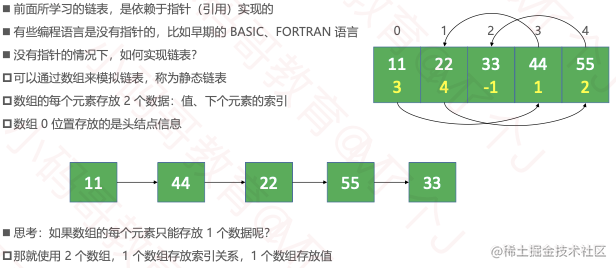### ArrayList能否進一步優化?

• int first: 儲存首元素的位置
• 刪除0號位, 改變first指標指向, first = 1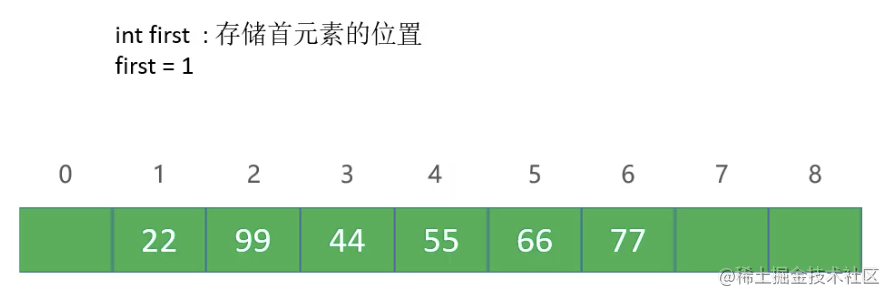### 雙向連結串列和動態陣列的區別

• 動態陣列: 開闢、銷燬記憶體空間的次數相對較少, 但可能造成記憶體空間浪費(可以通過縮容解決)
• 雙向連結串列: 開啟、銷燬空間的次數相對較多, 但不會造成記憶體空間的浪費

• 如果頻繁在`尾部`進行新增、刪除操作, 動態陣列、雙向連結串列`均可`選擇
• 如果頻繁在`頭部`進行新增、刪除操作, 建議選擇使用`雙向連結串列`
• 如果有頻繁的`在任意位置`新增、刪除操作, 建議選擇使用`雙向連結串列`
• 如果有頻繁的`查詢`操作, 建議選擇使用`動態陣列`

# 二、樹形結構, 樹

## 樹的基本概念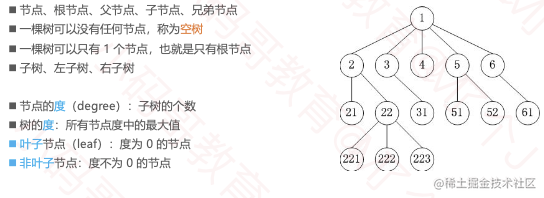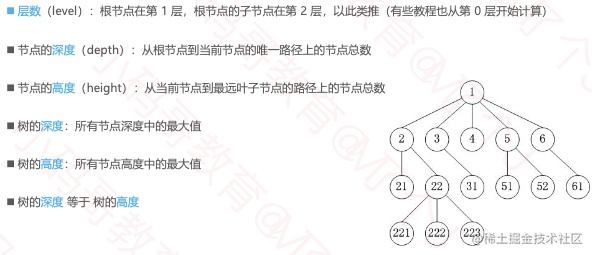## 二叉樹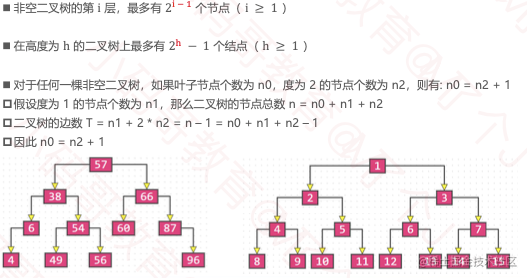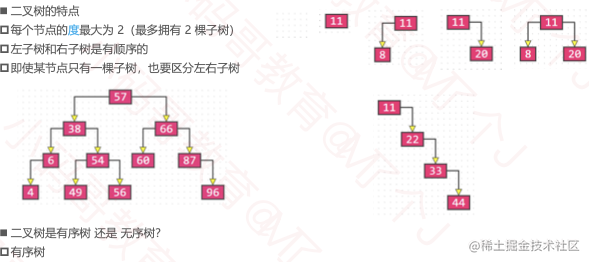• 二叉樹的性質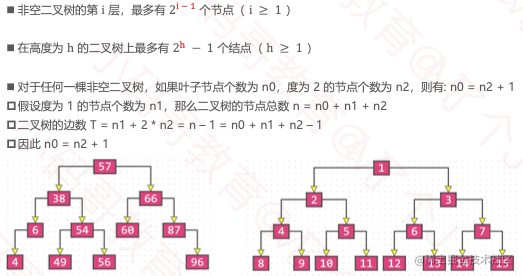### 真二叉樹(Proper Binary Tree)

• 所有節點的度要麼為0, 要麼為2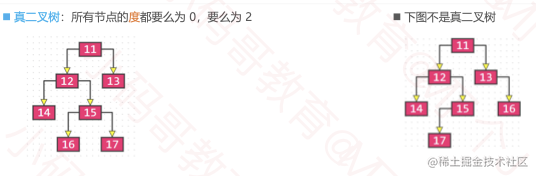### 滿二叉樹(Full Binary Tree)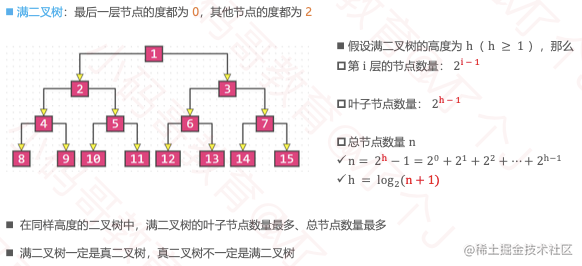### 完全二叉樹(Complete Binary Tree)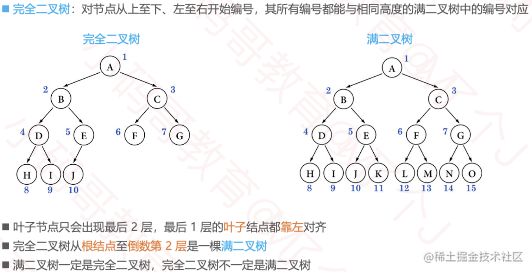- 完全二叉樹的性質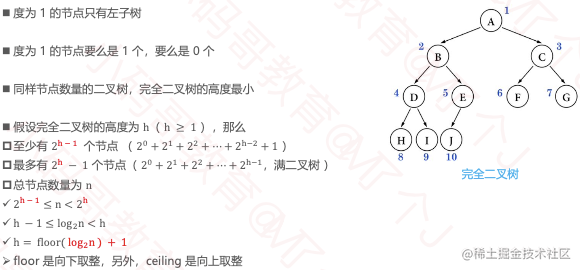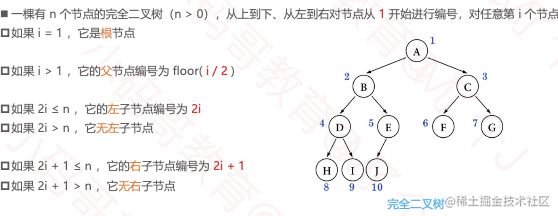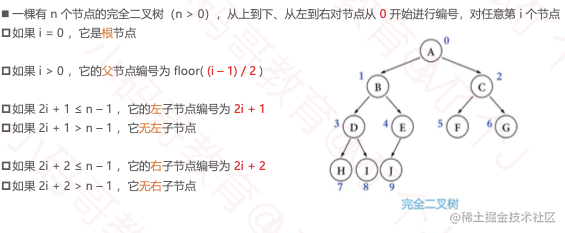• 下面就不是完全二叉樹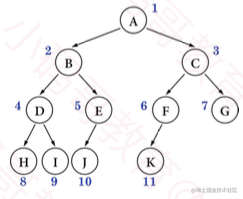### 常考點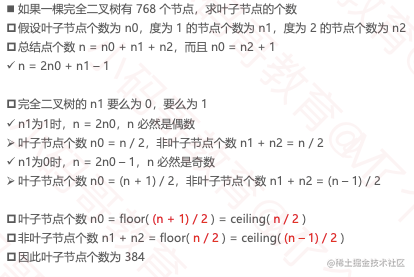### 二叉樹的遍歷

• 遍歷是資料結構中的常見操作, 把所有元素都訪問一遍
• 線性結構的遍歷比較簡單, 正序遍歷/逆序遍歷
• 根據節點訪問順序的也不同, 二叉樹的常見遍歷方式有四種
• 前序遍歷
• 中序遍歷
• 後序遍歷
• 層序遍歷

### 前序遍歷(Preorder Traversal)

• 訪問順序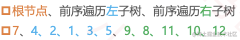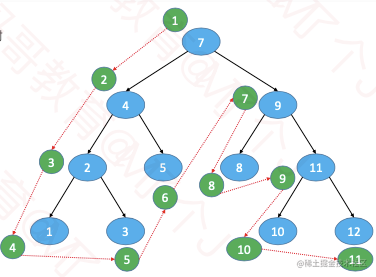### 中序遍歷(Inorder Traversal)

• 訪問順序
• 中序遍歷左子樹、根節點、中序遍歷右子樹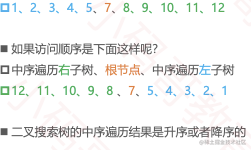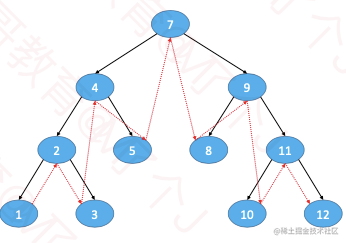### 後序遍歷 (Postorder Traversal)

• 訪問順序
• 後序遍歷左子樹、後序遍歷右子樹、根節點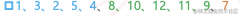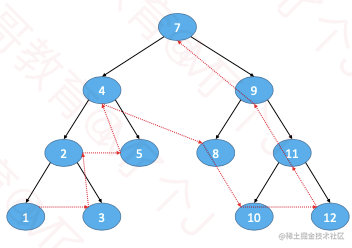### 層序遍歷 (Level Order Traversal)

• 訪問順序
• 從上到下、從左到右依次訪問每一個節點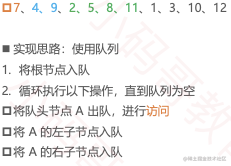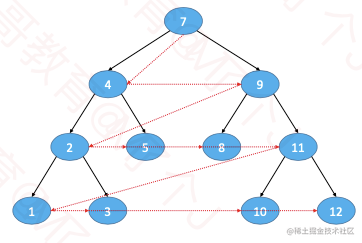### 力扣226. 翻轉二叉樹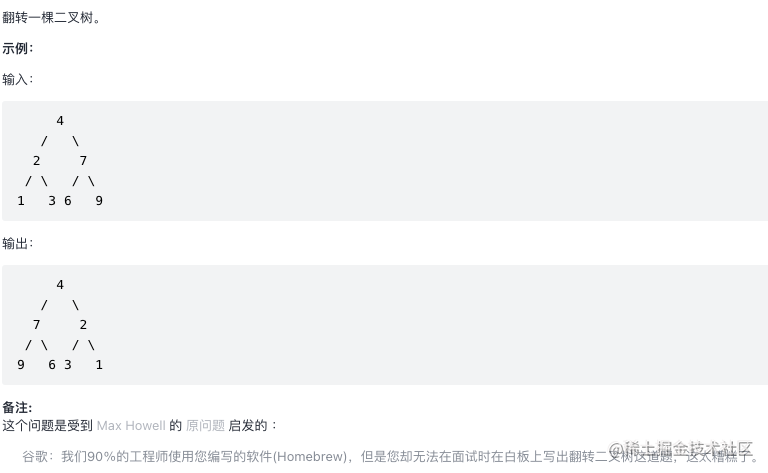``` /* * Definition for a binary tree node. * public class TreeNode { * int val; * TreeNode left; * TreeNode right; * TreeNode() {} * TreeNode(int val) { this.val = val; } * TreeNode(int val, TreeNode left, TreeNode right) { * this.val = val; * this.left = left; * this.right = right; * } * } / class Solution { public TreeNode invertTree(TreeNode root) { if (root == null) return null;

``````    // 前序遍歷, 先自己, 再左右
TreeNode tmpNode = root.left;
root.left = root.right;
root.right = tmpNode;

invertTree(root.left);
invertTree(root.right);

return root;
}
``````

}

class Solution { public TreeNode invertTree(TreeNode root) { if (root == null) return null;

``````    // 後序遍歷, 先左右, 再自己
invertTree(root.left);
invertTree(root.right);

TreeNode tmpNode = root.left;
root.left = root.right;
root.right = tmpNode;

return root;
}
``````

}

class Solution { public TreeNode invertTree(TreeNode root) { if (root == null) return null;

``````    // 中序遍歷
invertTree(root.left);

TreeNode tmpNode = root.left;
root.left = root.right;
root.right = tmpNode;

invertTree(root.left);

return root;
}
``````

} ```

# 力扣刷題

## 排序

### 氣泡排序

• 執行流程
• 從頭開始比較每一對相鄰元素, 如果第一個比第二個大, 就交換他們的位置
• 執行完一輪後, 最末尾的那個元素就是最大的元素
• 忽略曾經找到的最大元素, 重複執行第一步, 直到全部元素有序
• 最壞、平均時間複雜度: O(n²)

• 最好時間複雜度: O(n)
• 空間複雜度: O(1) ``` for (int end = array.length - 1; end > 0; end--) { for (int begin = 1; begin <= end; begin++) { if (cmp(begin, begin - 1) < 0) { swap(begin, begin - 1); } } }

// OC寫法 for (NSInteger end = result.count - 1; end > 0; end--) { for (NSInteger begin = 1; begin <= end; begin++) { NSInteger left = [result[begin-1] integerValue]; NSInteger right = [result[begin] integerValue]; if (left > right) { [result exchangeObjectAtIndex:begin-1 withObjectAtIndex:begin]; } } } `- 如果序列已經完全有序, 可以提前終止氣泡排序, 優化1` for (int end = array.length - 1; end > 0; end--) { boolean sorted = true; for (int begin = 1; begin <= end; begin++) { if (cmp(begin, begin - 1) < 0) { swap(begin, begin - 1); sorted = false; } } if (sorted) break; }

// OC寫法 NSMutableArray *result = [[NSMutableArray alloc] initWithArray:@[@1, @2, @5, @4, @3]]; for (NSInteger end = result.count - 1; end > 0; end--) {     BOOL sorted = YES;     for (NSInteger begin = 1; begin <= end; begin++) {         NSInteger left = [result[begin-1] integerValue];         NSInteger right = [result[begin] integerValue];         if (left > right) {             [result exchangeObjectAtIndex:begin-1 withObjectAtIndex:begin]; sorted = NO;         }     }     if (sorted) {         break;     } } `- 如果序列尾部已經區域性有序, 可以記錄最後一次交換的位置, 減少比較次數, 優化2` for (int end = array.length - 1; end > 0; end--) { int sortedIndex = 1; for (int begin = 1; begin <= end; begin++) { if (cmp(begin, begin - 1) < 0) { swap(begin, begin - 1); sortedIndex = begin; } } end = sortedIndex; }

// OC寫法 NSMutableArray *result = [[NSMutableArray alloc] initWithArray:@[@1, @2, @5, @4, @3]]; for (NSInteger end = result.count - 1; end > 0; end--) {     NSInteger sortedIndex = 1;     for (NSInteger begin = 1; begin <= end; begin++) {         NSInteger left = [result[begin-1] integerValue];         NSInteger right = [result[begin] integerValue];         if (left > right) {             [result exchangeObjectAtIndex:begin-1 withObjectAtIndex:begin];         }         sortedIndex = begin;     } end = sortedIndex; } ```

### 排序演算法的穩定性(Stability)

• 如果相等的2個元素, 在排序前後的相對位置保持不變, 那麼這是穩定的排序演算法
• 排序前: 5, 1, 3𝑎, 4, 7, 3𝑏
• 穩定的排序: 1, 3𝑎, 3𝑏, 4, 5, 7
• 不穩定的排序:1, 3𝑏, 3𝑎, 4, 5, 7
• 對自定義物件進行排序時, 穩定性會影響最終的排序效果
• 氣泡排序屬於穩定的排序演算法
• 稍有不慎, 穩定的排序演算法也能被寫成不穩定的排序演算法 ```for (NSInteger end = result.count - 1; end > 0; end--) { for (NSInteger begin = 1; begin <= end; begin++) { NSInteger left = [result[begin-1] integerValue]; NSInteger right = [result[begin] integerValue]; if (left >= right) {// > 不慎寫成 >= [result exchangeObjectAtIndex:begin-1 withObjectAtIndex:begin]; } } }```

### 原地演算法(In-place Algorithm)

• 什麼是原地演算法?
• 不依賴額外的資源或者依賴少數的額外資源, 僅依靠輸出來覆蓋輸入
• 空間複雜度為O(1)的都可以認為是原地演算法
• 非原地演算法, 稱為Not-in-place或者Out-of-place
• 氣泡排序屬於In-place

## 選擇排序

• 執行流程
• 從序列中找出最大的那個元素, 然後與最末尾的元素交換位置
• 執行完一輪後, 最末尾的那個元素就是最大的元素
• 忽略步驟1中已經找到的最大元素, 重複執行步驟1 ``` for (int end = array.length - 1; end > 0; end--) { int max = 0; for (int begin = 1; begin <= end; begin++) { if (cmp(max, begin) < 0) { max = begin; } } swap(max, end); }

// OC寫法 NSMutableArray *result = [[NSMutableArray alloc] initWithArray:@[@1, @2, @5, @4, @3]]; for (NSInteger end = result.count - 1; end > 0; end--) {     NSInteger max = 0;     for (NSInteger begin = 1; begin <= end; begin++) {         NSInteger begin = [result[begin] integerValue];         NSInteger max = [result[max] integerValue];         if (begin > max) {             max = begin;         }     } [result exchangeObjectAtIndex:end withObjectAtIndex:max]; } ``` - 選擇排序的交換次數要遠少於氣泡排序, 平均效能優於氣泡排序 - 最好、最壞、平均時間複雜度: O(n²), 空間複雜度: O(1), 屬於不穩定排序

## 堆排序

• 堆排序可以認為是對選擇排序的一種優化.
• 大頂堆

## 資料結構&演算法

### 掌握最常見的資料結構

• 陣列 @[@"12", @"34"]
• 優點: 查詢快index 遍歷方便
• 缺點: 增刪慢(找到第5個, 刪掉, 678前移) 只能儲存一種資料型別(新的tuple元組) 大小固定不方便擴容(int就固定了)
• 連結串列

• 優點: 增刪快(改變連結串列的指標)
• 缺點: 查詢特別麻煩(先從頭結點依次走下去)
• 雙向連結串列

• 指標指向前後資料
• @autoreleasepool
• 線性表

• 佇列
• queue, 裡面放任務
• 堆疊

• 棧, 壓棧, 先進後出, 頁面pop

### 樹

• 二叉樹, 遍歷, 順序, 二叉樹的翻轉
• 二叉樹既有連結串列的好處, 也有陣列的好處

### hash(散列表)

• 1-10 找到7, 遍歷
• 二分法 減少了時間複雜度
• 一次到位, 直接通過key找到value, 字典的底層就是hash
• 雜湊函式:f
• 1 - key - f(key) -> index=1
• 雜湊 把值1放到index=1的位置,
• 11 12 13 15 18
• 浪費資源, 雜湊函式沒有設計好
• f(key) = key - 10 = index 定義域key > 10
• 計算簡單 + 分佈均勻, 直接地址法, 資料分析法,
• 平方取中法(增大落差範圍, 導致衝突降低), 雜湊衝突
• 取餘法
• 9 13 14 15 16 18 % 10
• 9 3 4 5 6 8
• 資料分析 - 位運算 - index - 取值
• 設計出一個合理的, 分佈均勻的雜湊函式(雜湊函式)很難

#### 雜湊衝突

• 平方取中法
• 9 13 14 15 16 18
• 81 169 196 225 256 324 -- 雜湊衝突
• 繼續雜湊 - 再設計雜湊函式
• 判斷法 - 每一次移動
• 再平方法 - 減少你的操作
• 11 12 22 32
• 1 + 2^2 = 5
• 1 + 2^2 + 3^2 = 14
• 拉鍊法 -
• 11 12 22 32 42 52

### 常見的演算法題目

#### 1. 字串翻轉

• Hello,word =>
• Dorw,olleh
• 兩個變數記錄, 一個從前面走, 一個從末尾走, 換到最中間為止
• 移動 換值 指標

```void char_reverse(char *cha) { // 定義第一個字元 // 空間的首位就是指標的位置 char *begin = cha; // 定義一個末尾 char *end = cha + strlen(cha) - 1; while (begin < end) { // 核心邏輯 -- 換值, 移動 char jh_tmp = *begin; *(begin++) = *end; *(end--) = jh_tmp; } }```

#### 2. 翻轉連結串列

• 連結串列有個特性, 從頭開始, 沒有下標, 斷開非常容易
• 建立一個空的頭結點 ``` struct JHNode reverseList(struct JHNode head) { // 定義遍歷指標, 初始化為頭結點 struct JHNode p = head; // 反轉後的連結串列頭部 struct JHNode newH = NULL; // 遍歷連結串列 while (p != NULL) { // 記錄下一個節點 struct JHNode *temp = p->next; // 當前節點的next指向新連結串列頭部 p->next = newH; // 更改新連結串列頭部為當前節點 newH = p; // 移動p指標 p = temp; }

// 返回反轉後的連結串列頭結點 return newH; } ```

## 力扣刷題

### 力扣151. 翻轉字串裡的單詞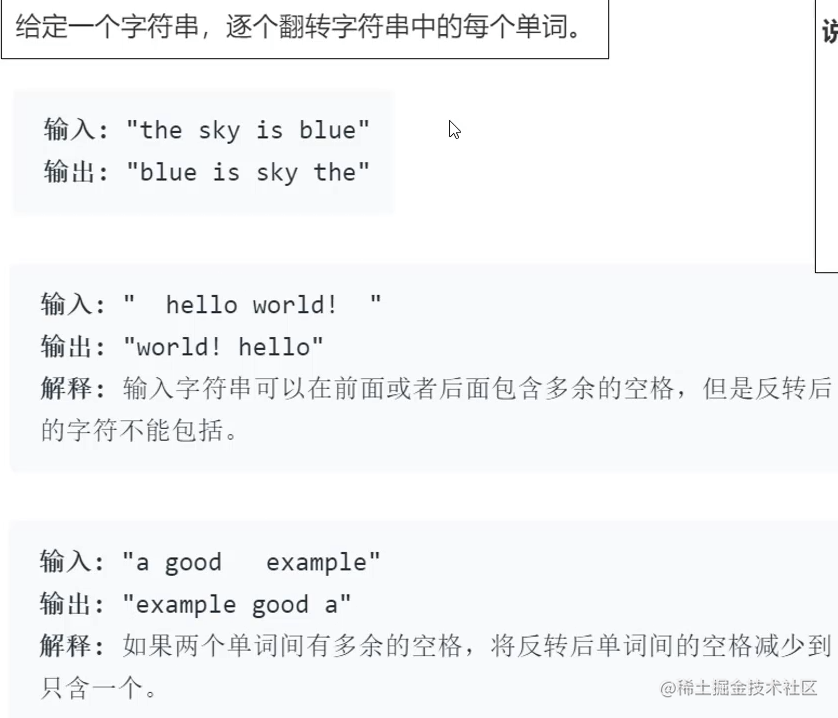1. 消除字串中的多餘空格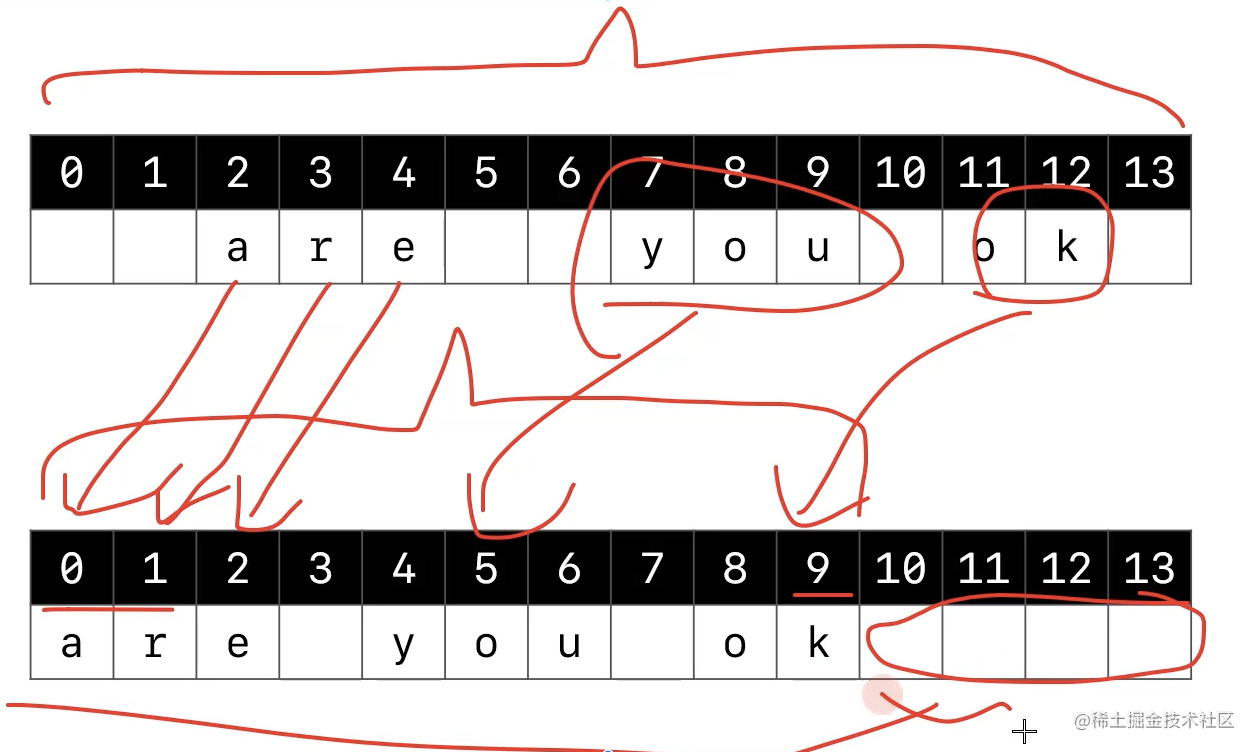2. 先0-10先逆序, 再逐個逆序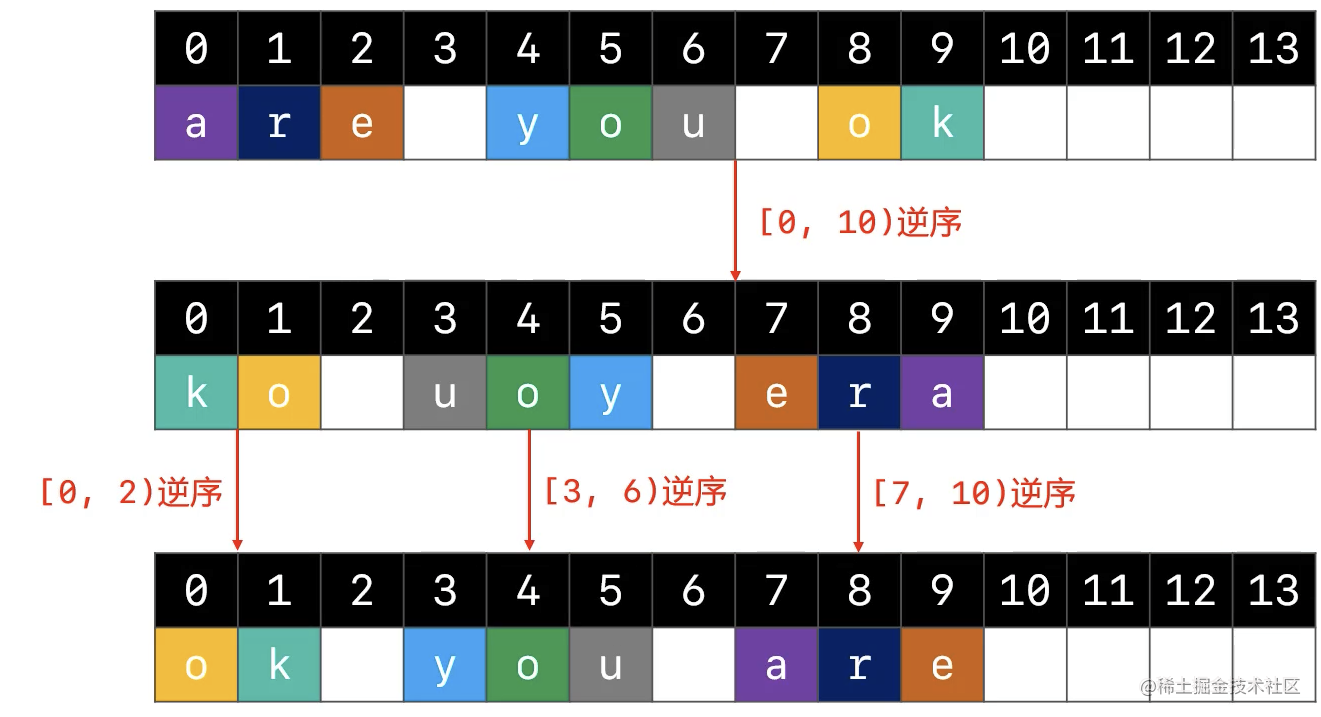``` public String reverseWords(String s) { // 容錯處理 if (s == null) return ""; char[] chars = s.toCharArray();

``````    // 1. 清除多餘的空格

// 字串最終的有效長度
int len = 0;
// 當前用來存放字元的位置
int cur = 0;
// 前一個字元是為空格字元
boolean preIsSpace = true;
// 遍歷字串
for (int i = 0; i < chars.length; i++) {
if (chars[i] != ' ') {// 當前字元chars[i]不是空格字元
chars[cur] = chars[i];
cur++;
preIsSpace = false;// cur指標++移動後, 前一個字元不是空格字元
} else if (preIsSpace == false) {// chars[i]是空格字元 且 前一個字元chars[i - 1]不是空格
chars[cur] = ' ';
cur++;
preIsSpace = true;
}
}
// 遍歷結束後, 最終的 前一個字元是空格字元
len = preIsSpace ? (cur - 1) : cur;
if (len <= 0) return "";

// 2. 對整個字串進行逆序
reverse(chars, 0, len);

// 3. 對每一個單詞進行逆序
// 前一個空格字元的位置(在-1位置有個假想的哨兵, 就是要一個假想的空格符)
int preSpaceIdx = -1;
for (int i = 0; i < len; i++) {
if (chars[i] != ' ') continue;
// 遍歷到空格字元
reverse(chars, preSpaceIdx + 1, i);
preSpaceIdx = i;
}

// 4. 對最後一個單詞進行逆序
reverse(chars, preSpaceIdx + 1, len);

return new String(chars, 0, len);
}

// 將[li, ri)範圍內的字串進行逆序
private void reverse(char[] chars, int li, int ri) {
ri--;
while (li < ri) {
char tmp = chars[li];
chars[li] = chars[ri];
chars[ri] = tmp;
li++;
ri--;
}
}
``````

} ```

### 力扣3. 無重複字元的最長子串

• 給定一個字串, 請你找出其中不含有重複字元的最長子串的長度.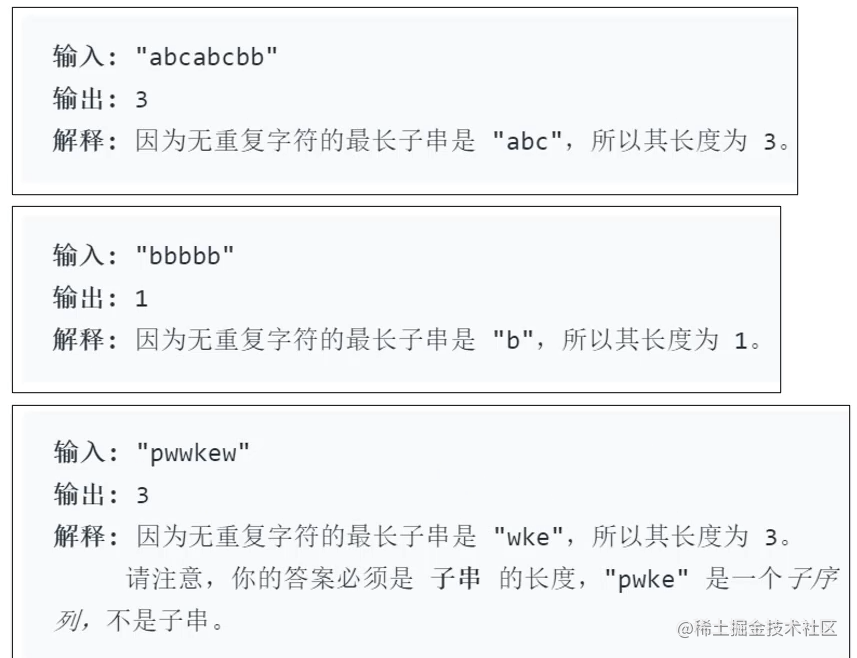• 有點動態規劃的感覺

• 最長無重複字串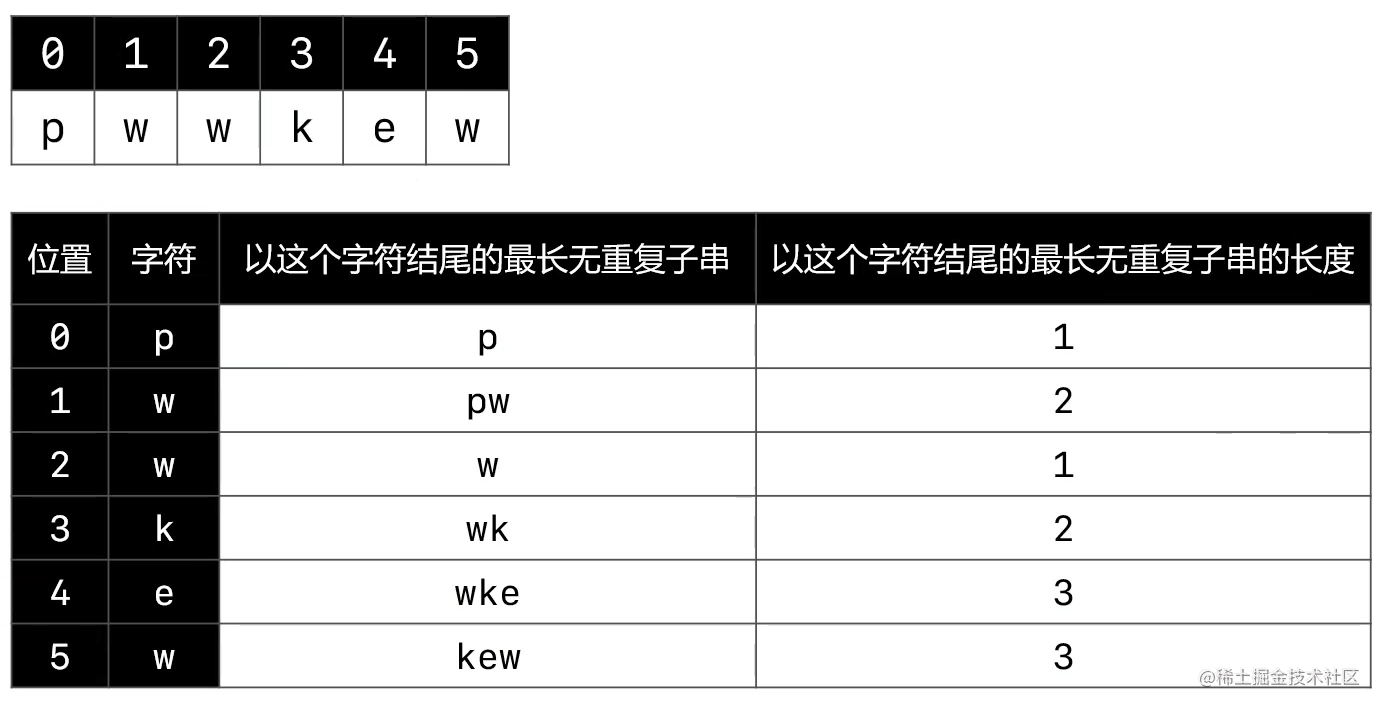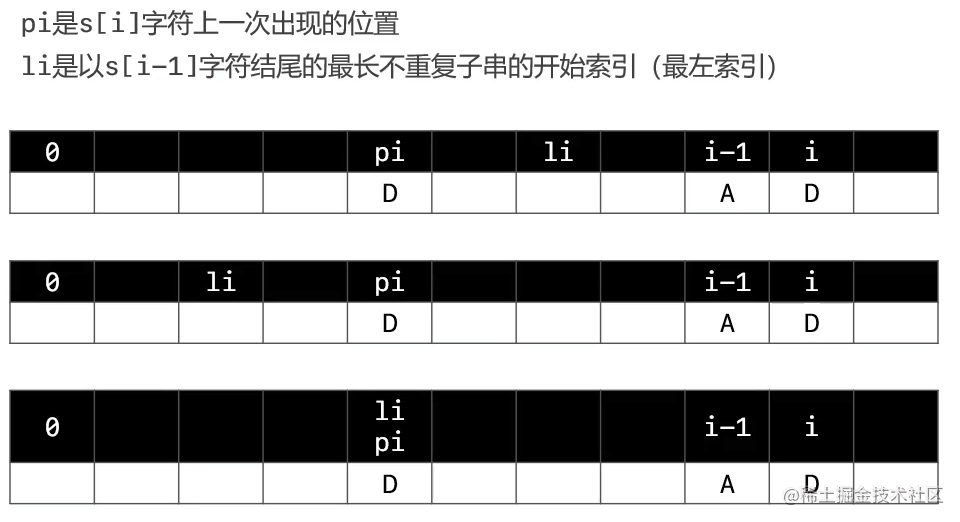- 雜湊表技術 ``` public int lengthOfLongestSubstring(String s) { if (s == null) return 0; char[] chars = s.toCharArray(); if (chars.length== 0) return 0;

``````    // 用來儲存每一個字元上一次出現的位置
Map<Character, Integer> prevIdxes = new HashMap<>();
// 掃描過零號位置
prevIdxes.put(chars, 0);

/**
// 小寫字母26陣列優化, ASCII 128, 假設是單位元組字元
// 用來儲存每一個字元上一次出現的位置
int[] prevIdxed = new int;
for (int i = 0; i < prevIdxed.length; i++) {
prevIdxes[i] = -1;
}
prevIdxes[chars] = 0;
*/

// 以i - 1位置字元結尾的最長不重複字串的開始索引(最左索引)
int li = 0;
int max = 1;
for (int i = 1; i < chars.length; i++) {
// i位置字元上一次出現的位置
Integer pi = prevIdxes.get(chars[i]);
if (pi != null && li <= pi) {
li = pi +1;
}

// 儲存這個字元出現的位置
prevIdxes.put(chars[i], i);

/**
// i位置字元上一次出現的位置
int pi = prevIdxes[chars[i]];
if (li <= pi) {
li = pi + 1;
}
// 儲存這個字元出現的位置
prevIdxes[chars[i]] = i;
*/

// 求出最長不重複子串的長度
max = Math.max(max, i - li + 1);

}

return max;
}
``````

```

## 動態規劃(Dynamic Programming)

• 動態規劃, 建成DP
• 是求解最優化問題的一種常用策略
• 通常的使用套路(一步一步優化)
• 暴力遞迴(自頂向下, 出現了重疊子問題)
• 記憶化搜尋(自頂向下)
• 遞推(自底向上)

### 力扣劍指Offer47. 禮物的最大價值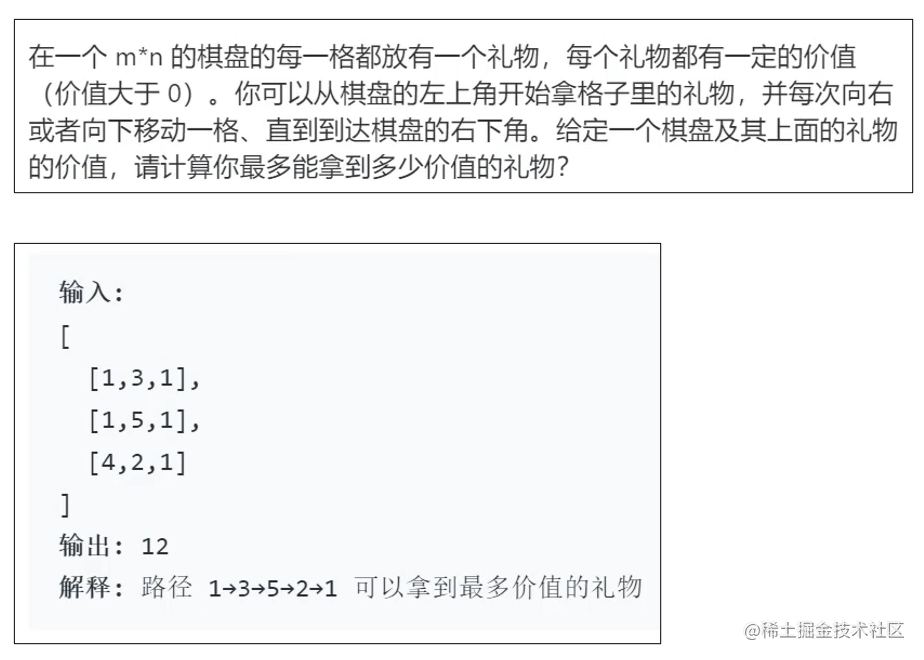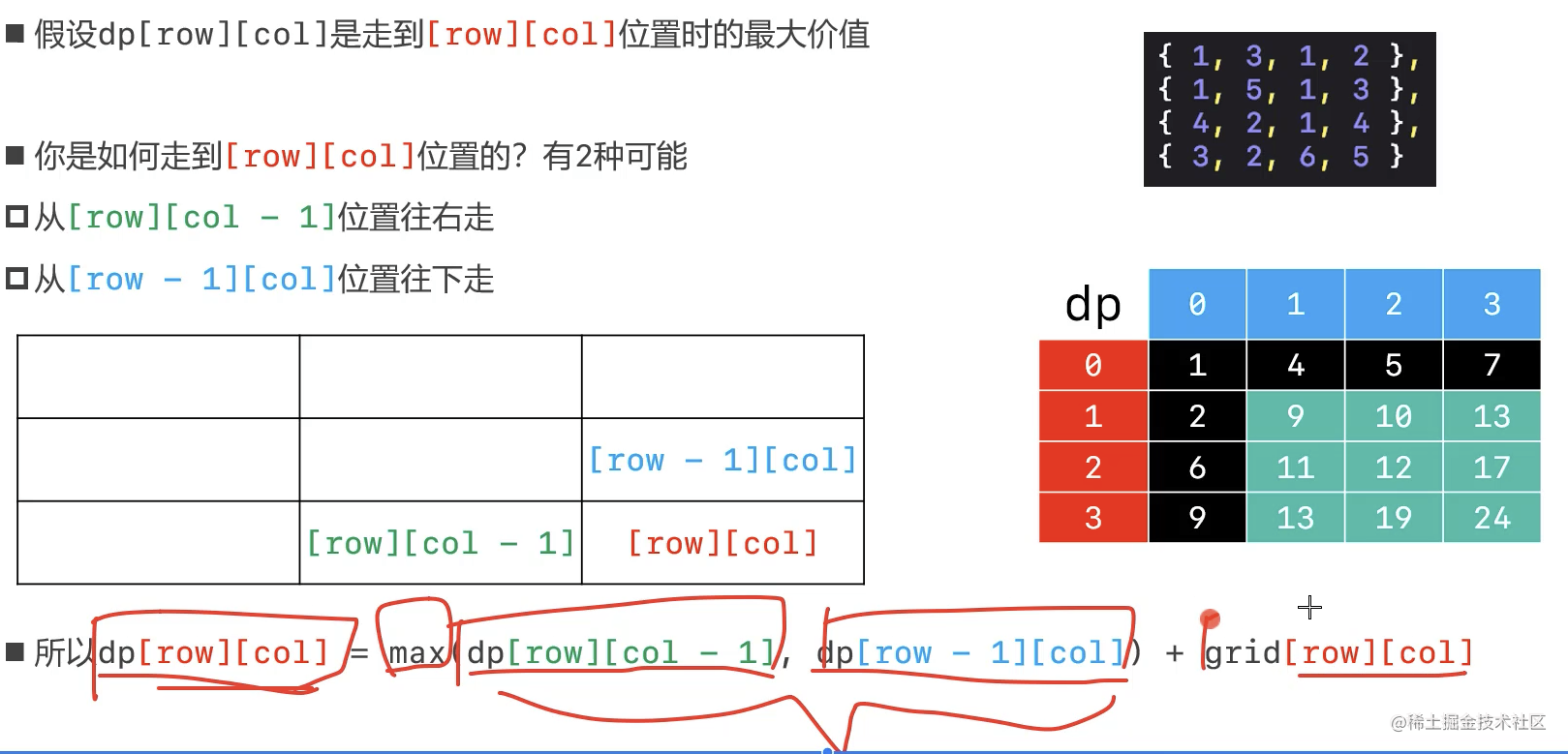``` public int maxValue(int[][] grid) { // 行數 int rows = grid.length; // 列數 int cols = grid.length;

``````    // 動態規劃, 建立行列數的二維陣列
int[][] dp = new int[rows][cols];

// 確定初始位置
dp = grid;

// 第0行, 遍歷列, 給動態規劃二維陣列賦值0行全部列值
for (int col = 1; col < cols; col++) {
dp[col] = dp[col - 1] + grid[col];
}

// 第0列, 遍歷行, 給動態規劃二維陣列賦值0列全部行值
for (int row = 1; row < rows; row++) {
dp[row] = dp[row - 1] + grid[row];
}

// 全部遍歷, 對比哪個大, 賦值其他位置
for (int row = 1; row < rows; row++) {
for (int col = 1; col < cols; col++) {
dp[row][col] =  Math.max(dp[row - 1][col], dp[row][col - 1]) + grid[row][col];
}
}

return dp[rows - 1][cols - 1];
}
``````

```

### 力扣121. 買賣股票的最佳時機

• 給定一個數組 prices ，它的第 i 個元素 prices[i] 表示一支給定股票第 i 天的價格。
• 你只能選擇 某一天 買入這隻股票，並選擇在 未來的某一個不同的日子 賣出該股票。設計一個演算法來計算你所能獲取的最大利潤。
• 返回你可以從這筆交易中獲取的最大利潤。如果你不能獲取任何利潤，返回 0 。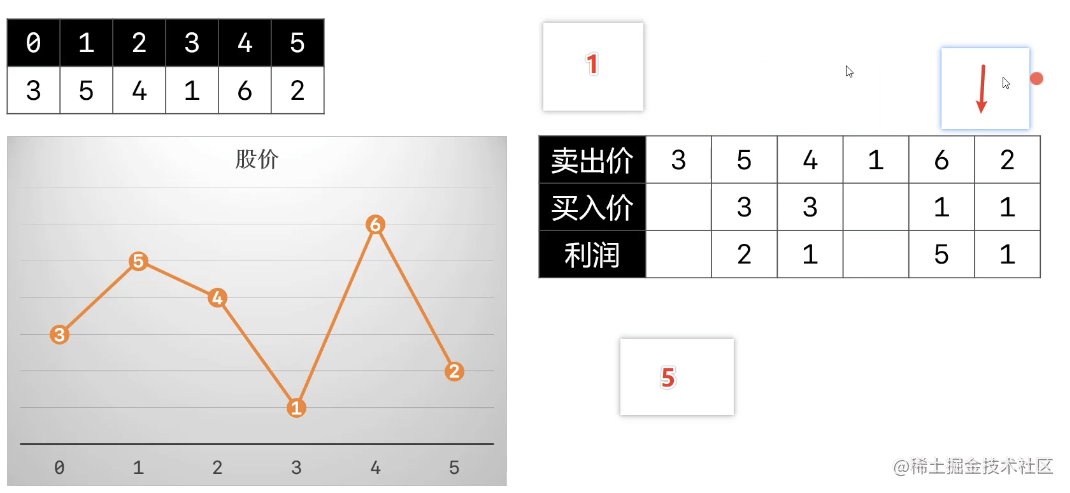``` public int maxProfit(int[] prices) { if ( prices == null || prices.length == 0) return 0;

``````// 當前掃描過的最小股票價格, 預設0號位
int minPrice = prices;
// 當前掃描過的最大利潤
int maxProfit = 0;

// 從1號位開始遍歷所有價格
for (int i = 1; i < prices.length; i++) {
if (prices[i] < minPrice) {// 最小股票價格小於第i天的價格
minPrice = prices[i];
} else {// 有最小股票價格時以第i天的價格賣出股票, 對比獲得最大利潤
maxProfit = Math.max(maxProfit, prices[i] - minPrice);
}
}

return maxProfit;
``````

} ```

### 力扣72. 編輯距離 困難

• 編輯距離演算法被資料科學家廣泛應用, 是用作機器翻譯和語音識別評價標準的基本演算法.
• 給定兩個單詞word1和word2, 計算出將word1轉換成word2所使用的最少運算元.
• 你可以對一個單詞進行如下三種操作:
• 插入一個字元
• 刪除一個字元
• 替換一個字元 ```輸入: word1 = "horse", word2 = "ros" 輸出: 3 解釋: horse -> rorse (將'h' 替換為 'r') rorse -> rose (刪除 'r') rose -> ros (刪除 'e')```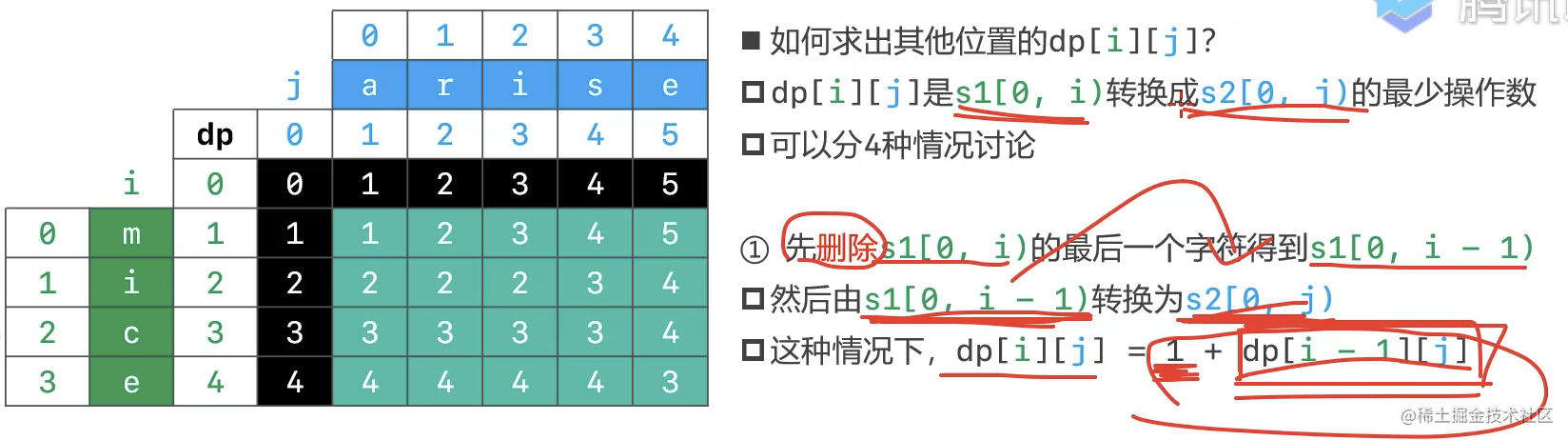• 前兩種情況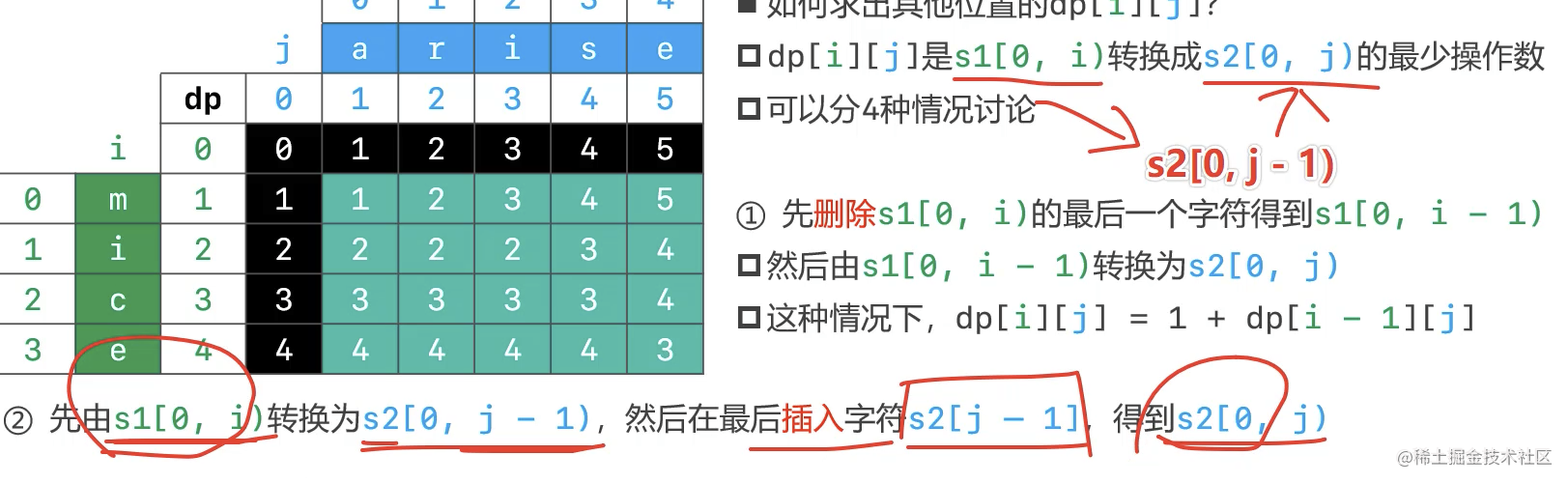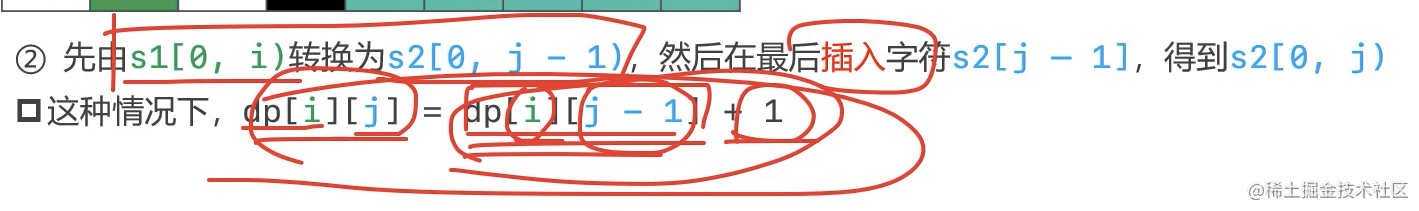• 第三種情況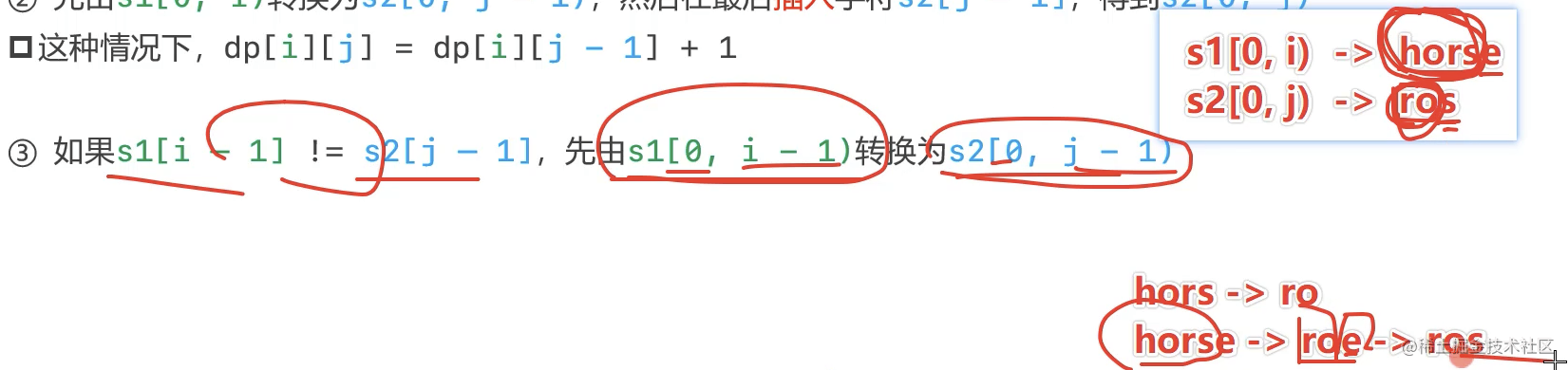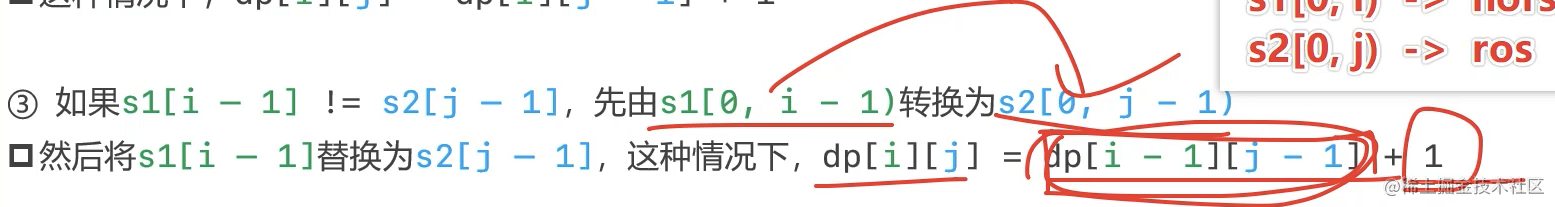• 第四種情況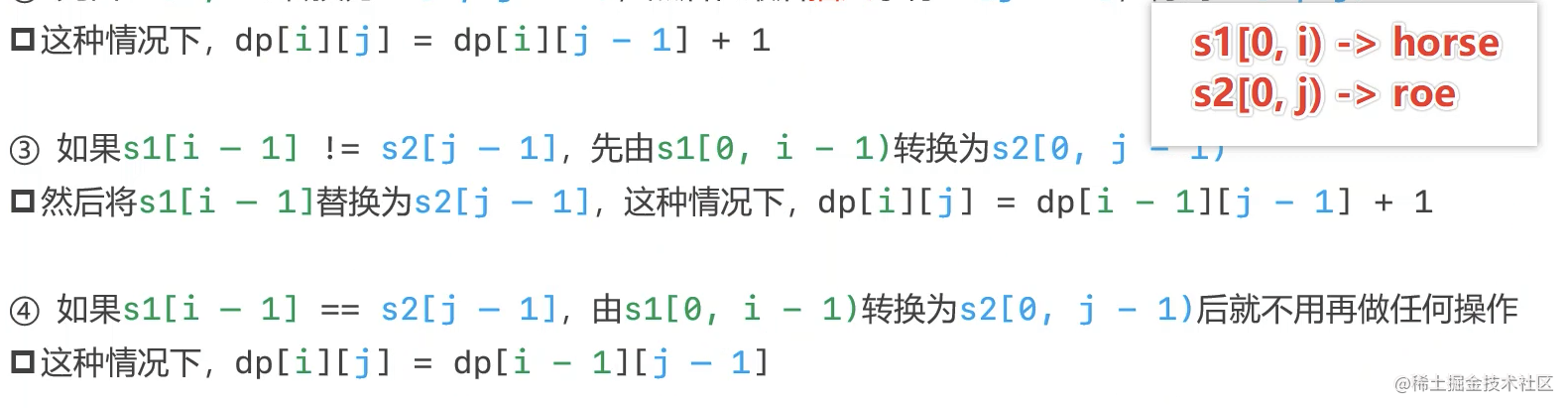• 三條路: 從上面、從左面、從左上角

• 挑一個最小的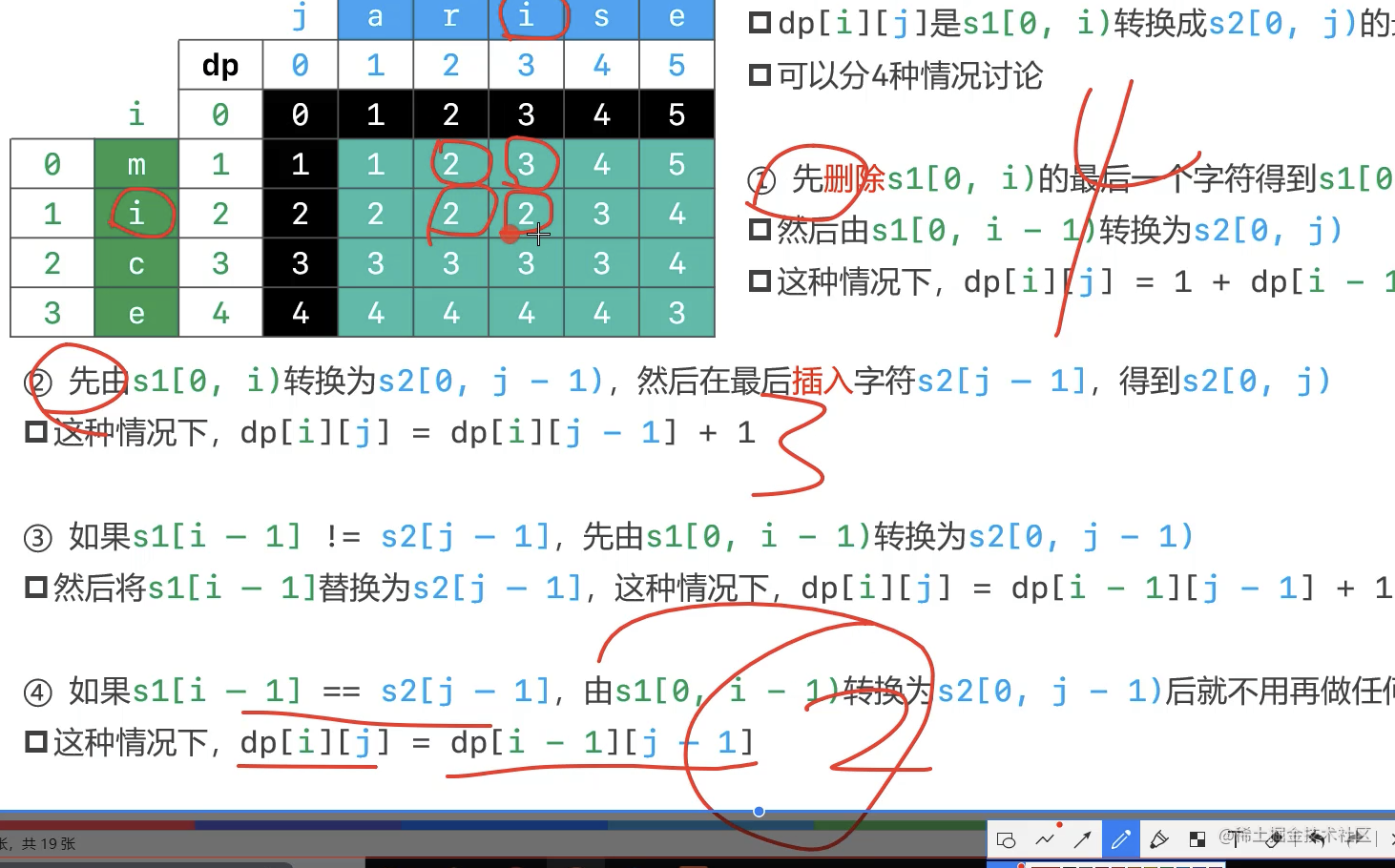``` public int minDistance(String word1, String word2) { if (word1 == null || word2 == null) return 0; char[] cs1 = word1.toCharArray();// 行 char[] cs2 = word2.toCharArray();// 列 int[][] dp = new int[cs1.length + 1][cs2.length + 1]; dp = 0;

``````    // 第0列, 最短路徑就是當前行字串的長度
for (int i = 1; i <= cs1.length; i++) {
dp[i] = i;
}

// 第0行, 最短路徑就是當前列字串的長度
for (int j = 1; j <= cs2.length; j++) {
dp[j] = j;
}

// 其他行其他列的最短路徑
for (int i = 1; i <= cs1.length; i++) {
for (int j = 1; j <= cs2.length; j++) {
// 可能的三條計算路徑: 從上面、從左面、從左上角
int top = dp[i - 1][j] + 1;// 上一行, 同一列
int left = dp[i][j - 1] + 1;// 上一列, 同一行
int leftTop = dp[i - 1][j - 1];

// 如果最後一個字串不相等, 需要多一步替換操作
if (cs1[i - 1] != cs2[j - 1]) {
leftTop++;
}

dp[i][j] = Math.min(Math.min(top, left), leftTop);
}
}

return dp[cs1.length][cs2.length];
}
``````

``` - 難點在狀態定義、狀態轉移方程, 怎麼推匯出下一個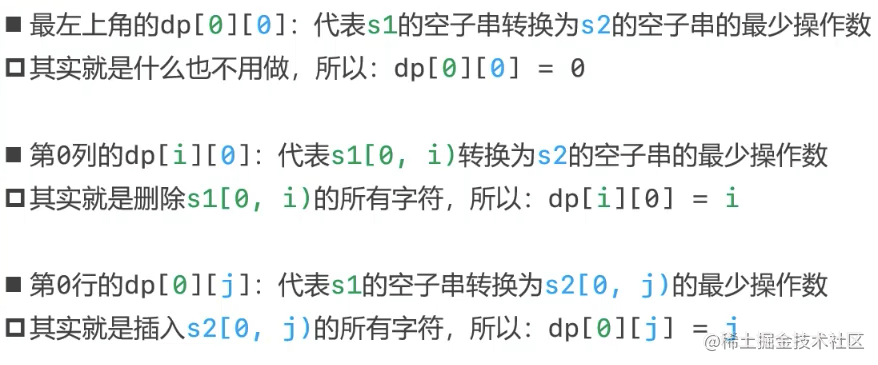• 二維陣列可以優化成一維陣列

### 力扣5. 最大回文子串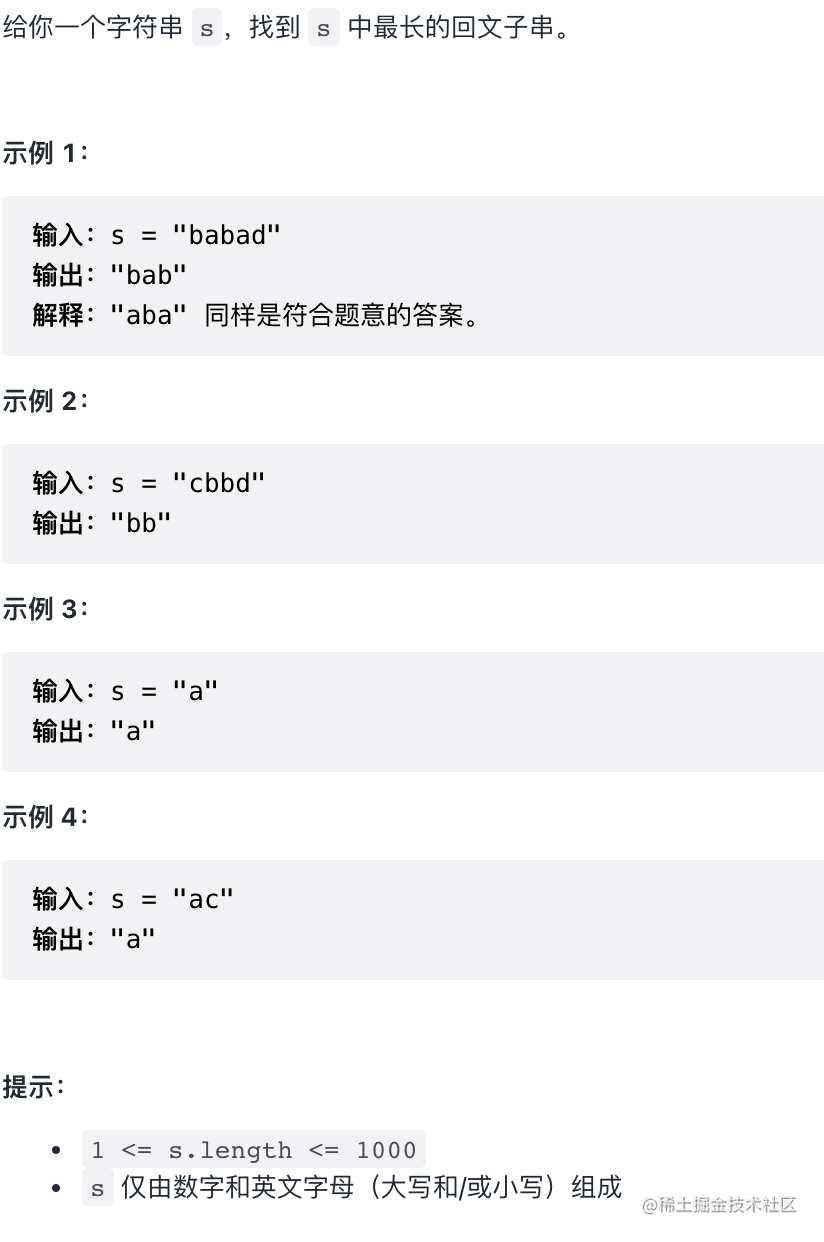#### 暴力法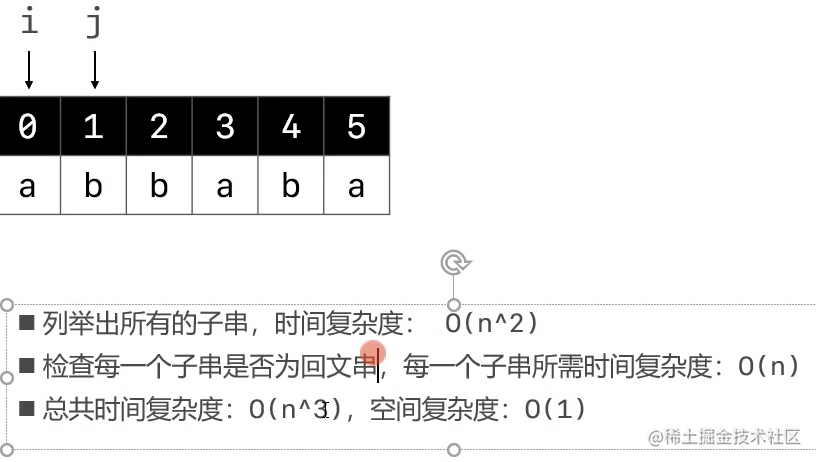#### 動態規劃法

• 對比暴力法, 其實是暴力法的優化把時間複雜度優化從 n^3 到了 n^2
• 空間複雜度O(n^2), 空間換時間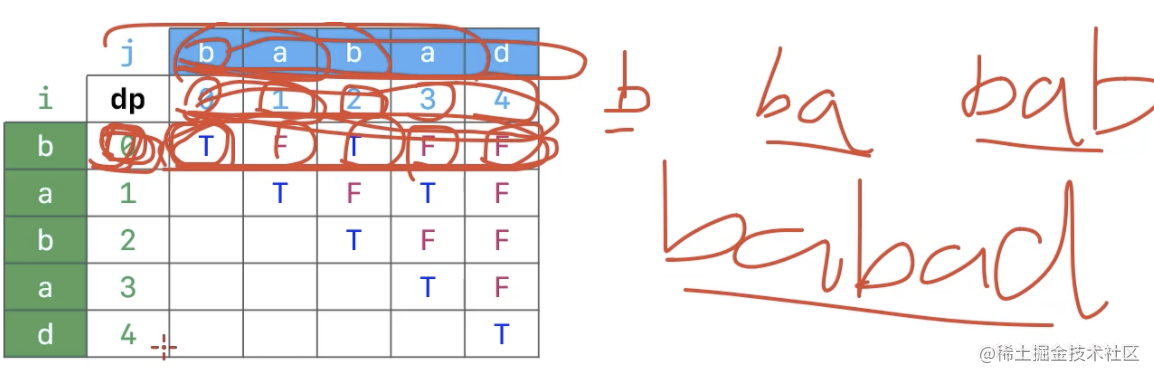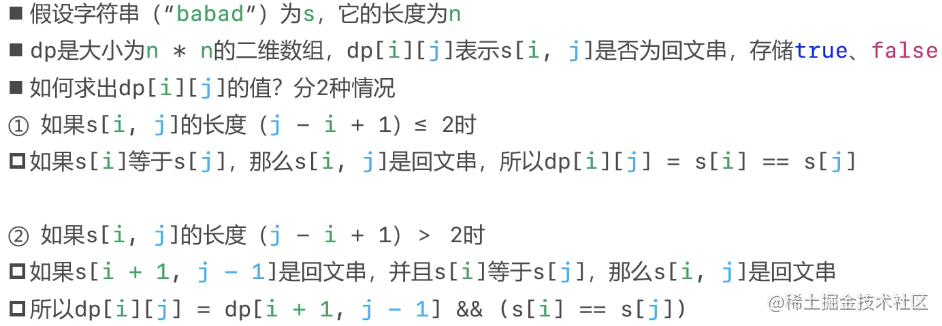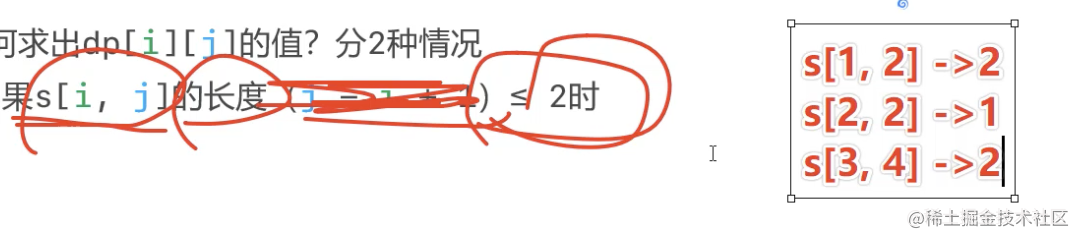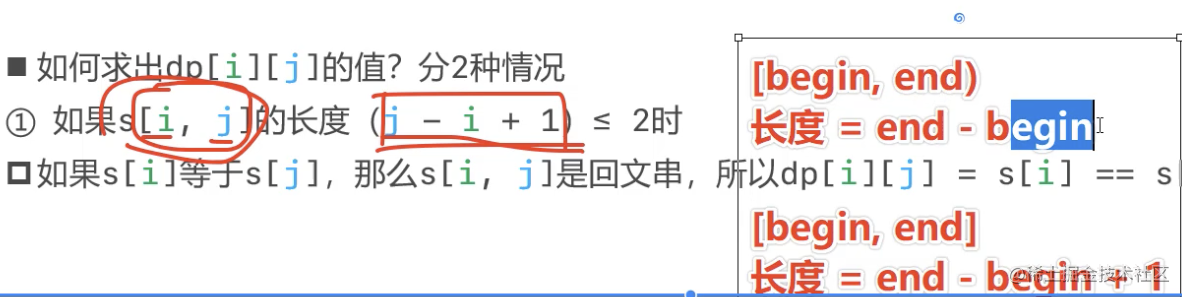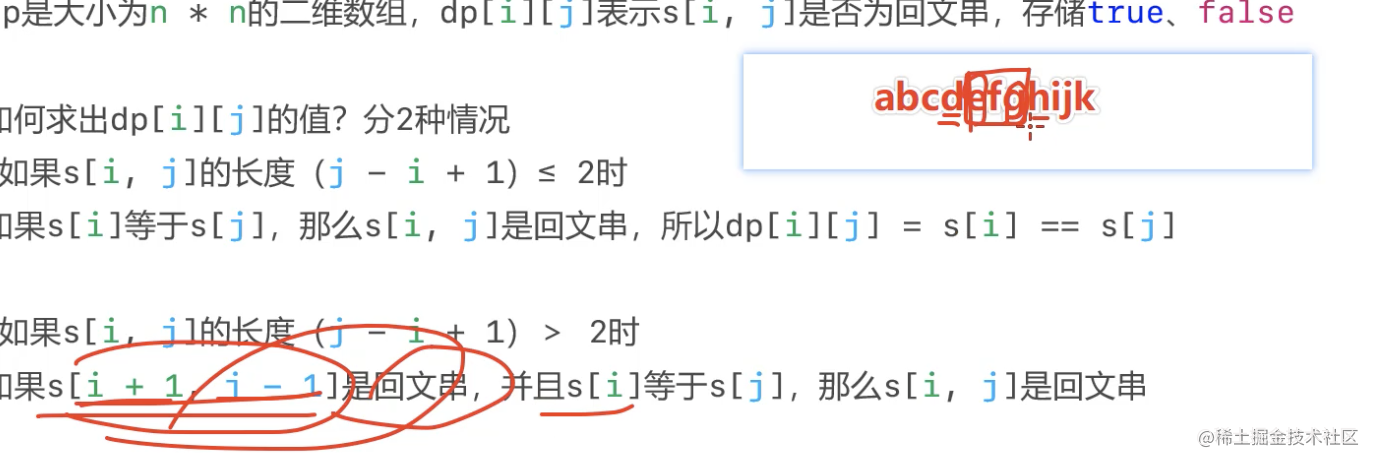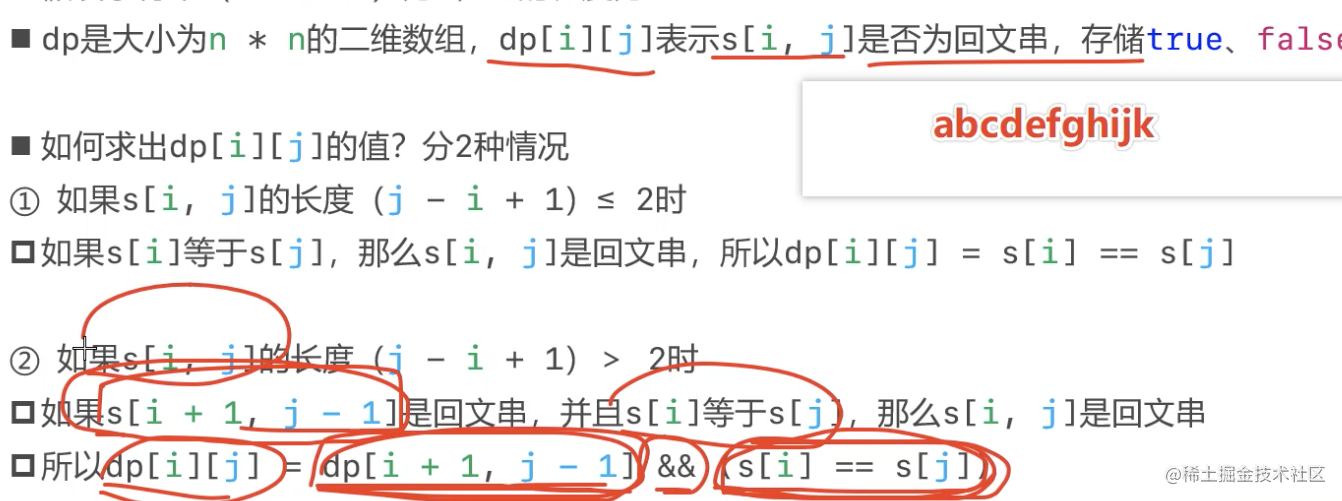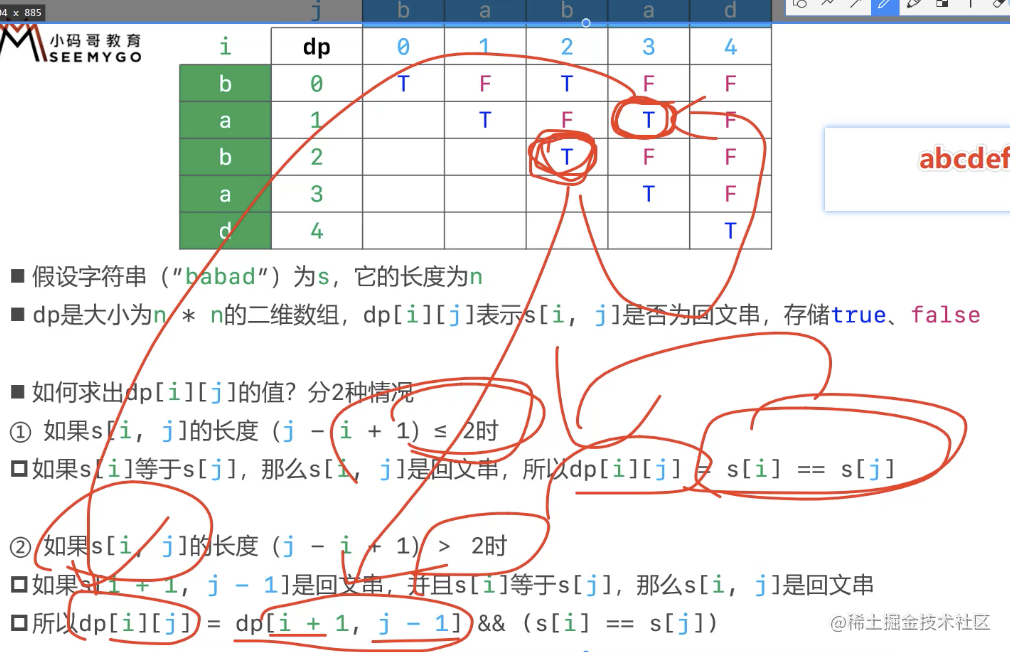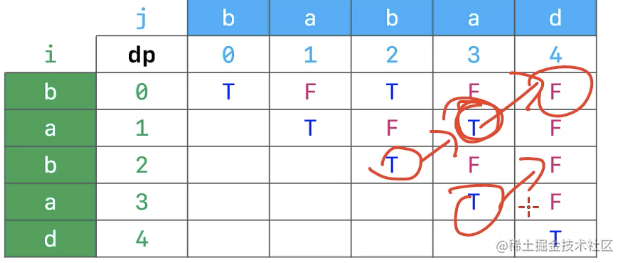- 從下到上, 從做到右 ``` public String longestPalindrome(String s) { if (s == null) return null; char[] cs = s.toCharArray(); if (cs.length == 0) return s;

``````    // 最長迴文子串的長度(至少是1)
int maxLength = 1;
// 最長迴文子串的開始索引
int begin = 0;

// 建立布林型別的動態規劃二維陣列
boolean[][] dp = new boolean[cs.length][cs.length];

// 從下到上 (i由大到小)
for (int i = cs.length - 1; i >= 0; i--) {
// 從左到右(j有小到大)
for (int j = i; j < cs.length ; j++) {
// cs[i, j]字串的長度
int length = j - i + 1;

// 兩種情況
/**
1. 字串長度 <= 2時, 長度為1或2, cs[i]字元等於cs[j]字元, 那麼cs[i, j]是迴文串,
此時dp[i][j] = cs[i] == cs[j]

2. 字串長度 > 2時, 如果動態規劃表當前字串的左下方cs[i + 1, j - 1]是迴文串 aaadefed,
且cs[i]字元等於cs[j]字元, 那麼cs[i, j]是迴文串
此時dp[i][j] = dp[i + 1][j - 1] && ()cs[i] == cs[j])
*/
dp[i][j] = (cs[i] == cs[j]) && (length <= 2 || dp[i + 1][j - 1]);

// 當前cs[i, j]是迴文子串 且 長度大於儲存的最大長度, 重新賦值
if (dp[i][j] && (length > maxLength)) {
maxLength = length;
begin = i;
}
}
}
return new String(cs, begin, maxLength);
}
``````

```

### 給定一個三角形 `triangle`, 找出自頂向下的最小路徑和.

• 每一步只能移動到下一行中相鄰的結點上. 相鄰的結點 在這裡指的是 下標上一層結點下標 相同或者等於 上一層結點下標 + 1 的兩個結點. ```輸入: triangle = [, [3,4], [6,5,7], [4,1,8,3]] 輸出: 11 解釋: 如下面簡圖所示```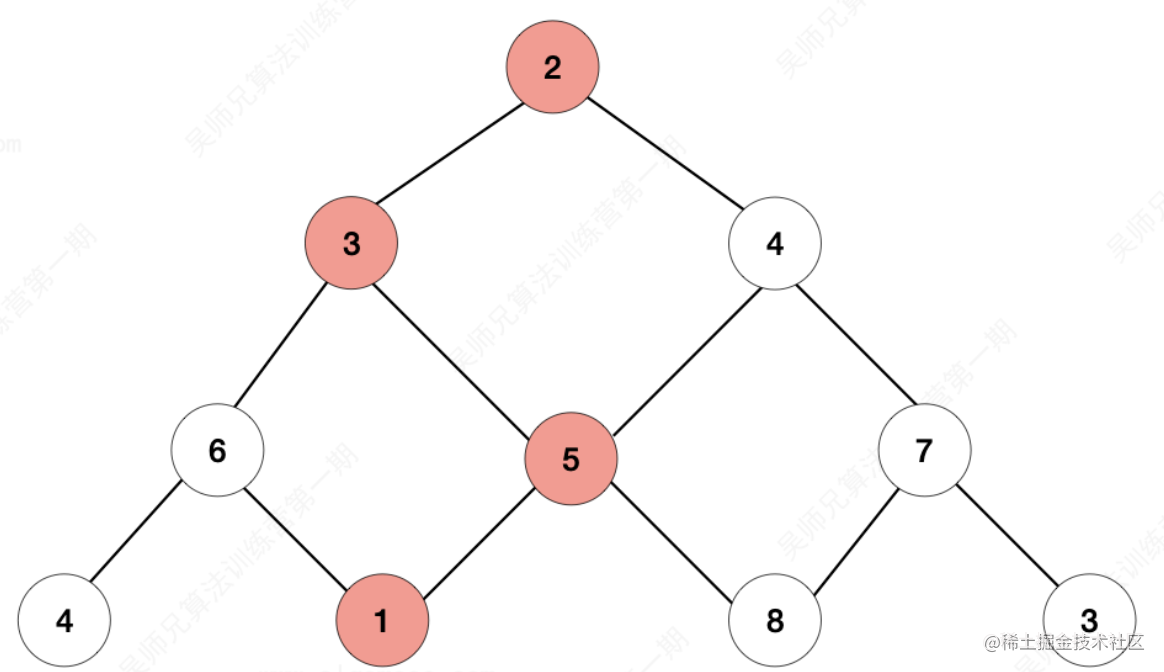- 自頂向下的最小路徑和為11(即, 2 + 3 + 5 + 1 = 11). - 解法採用: 1. 從上往下的動態規劃 2. 從下往上的動態規劃 3. 從下往上的動態規劃(使用一維陣列)

• 思路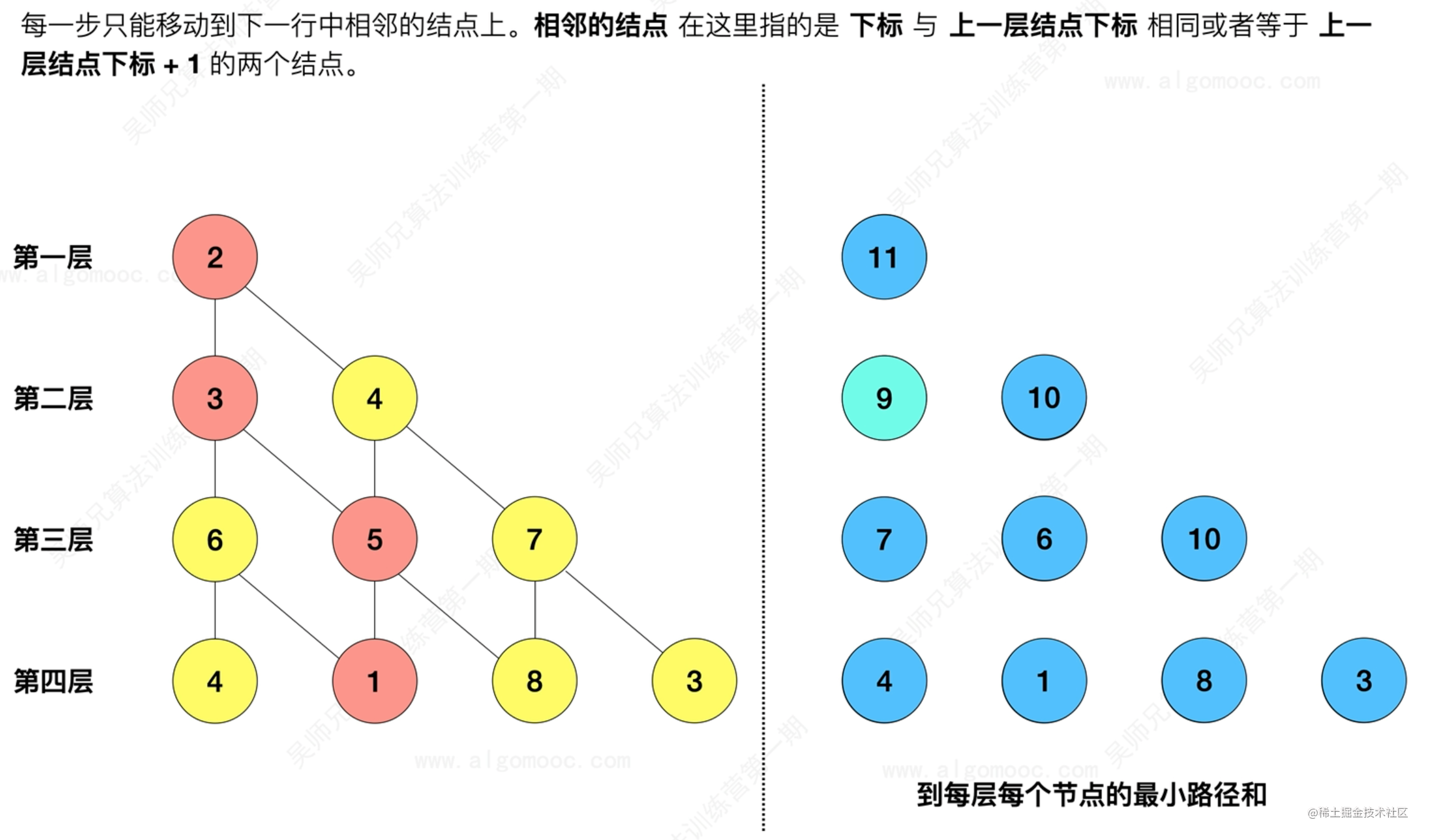• 儲存的是到達第i+1層各個結點的最小路徑之和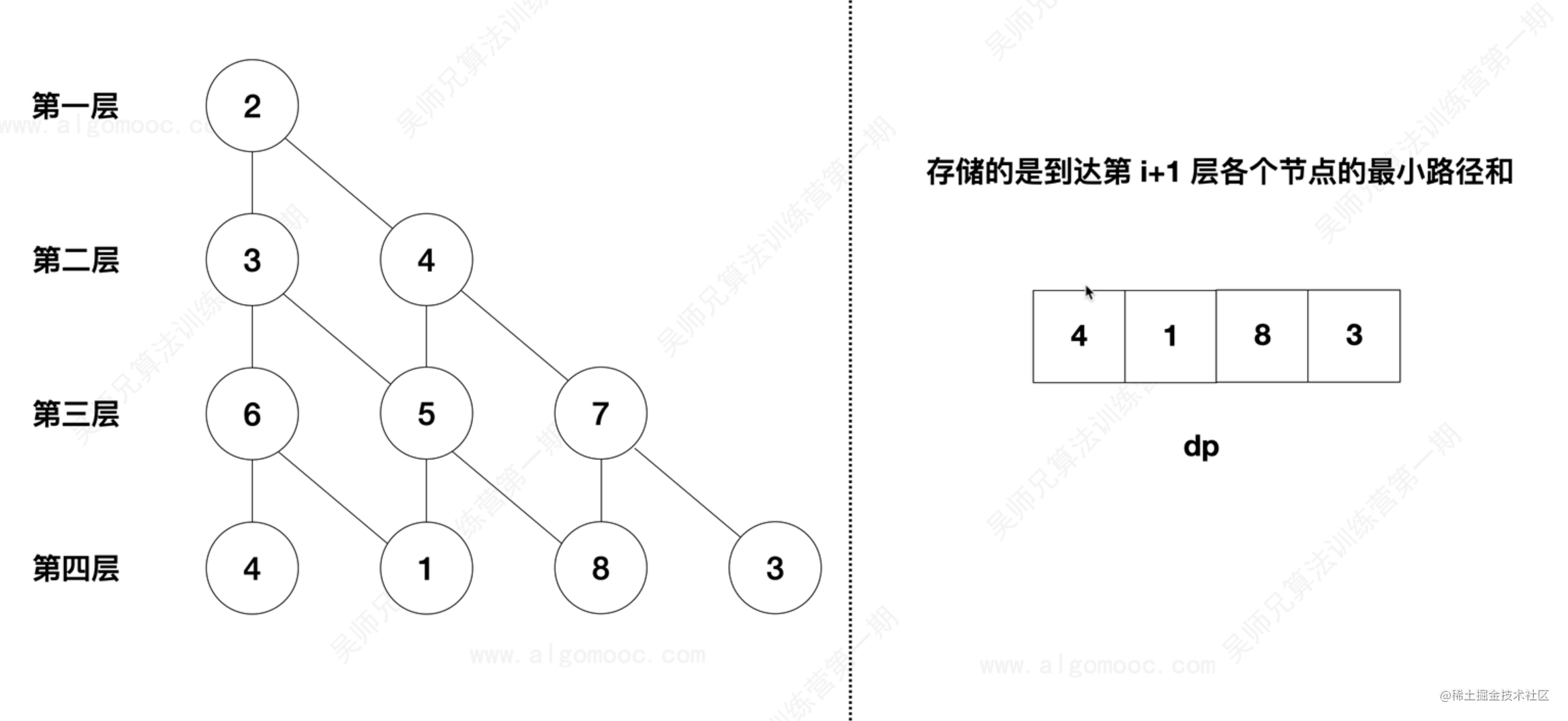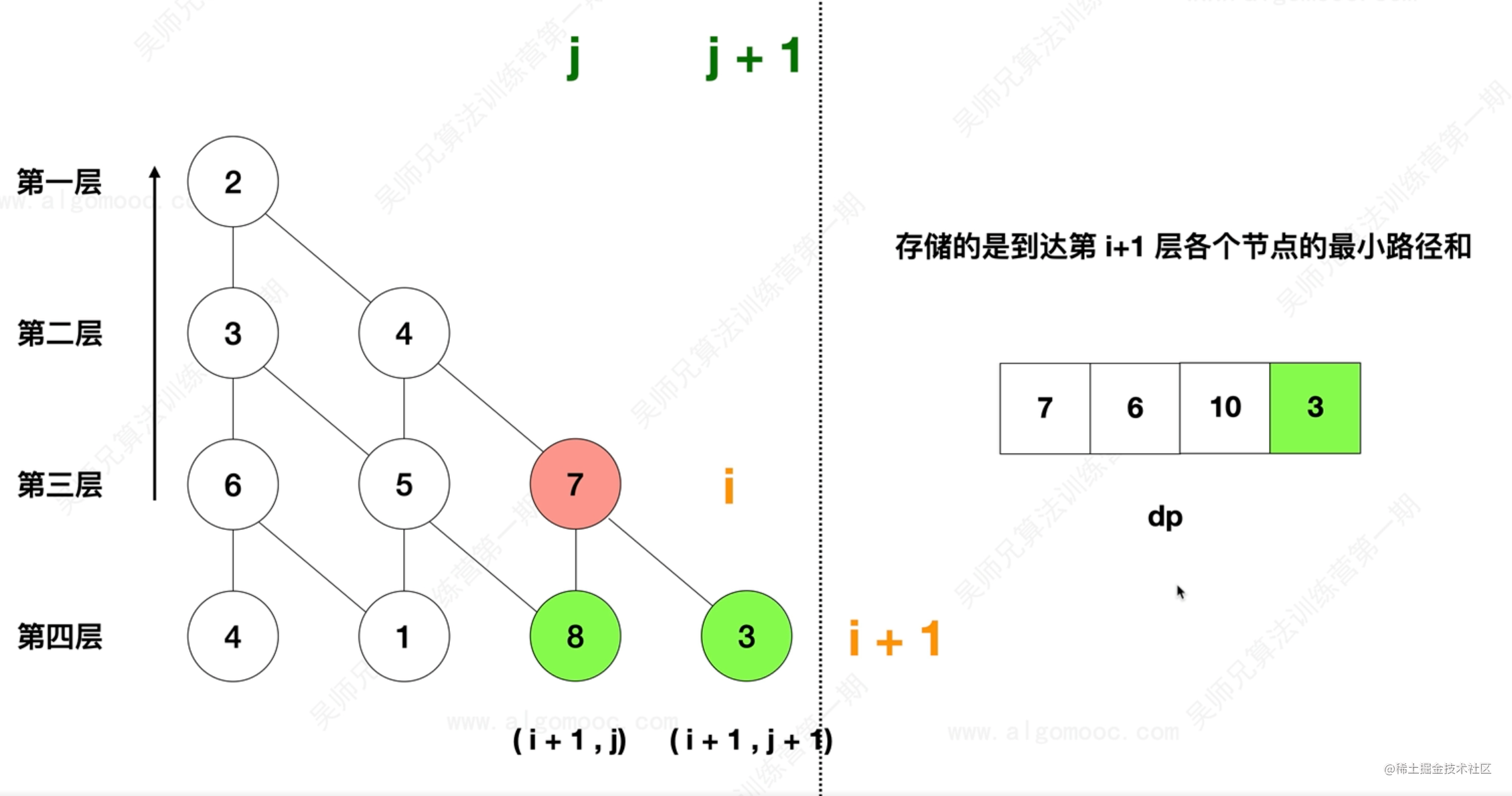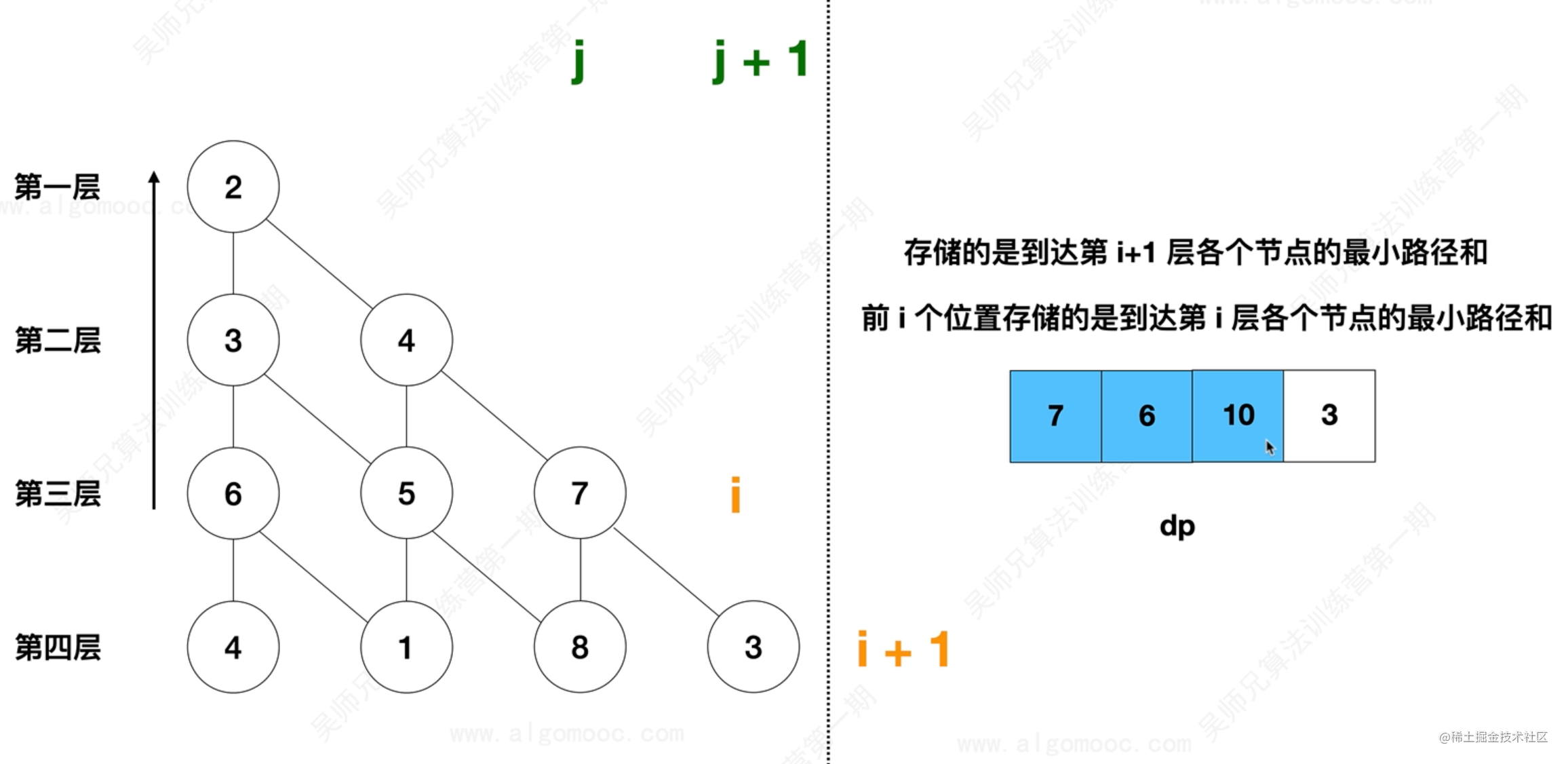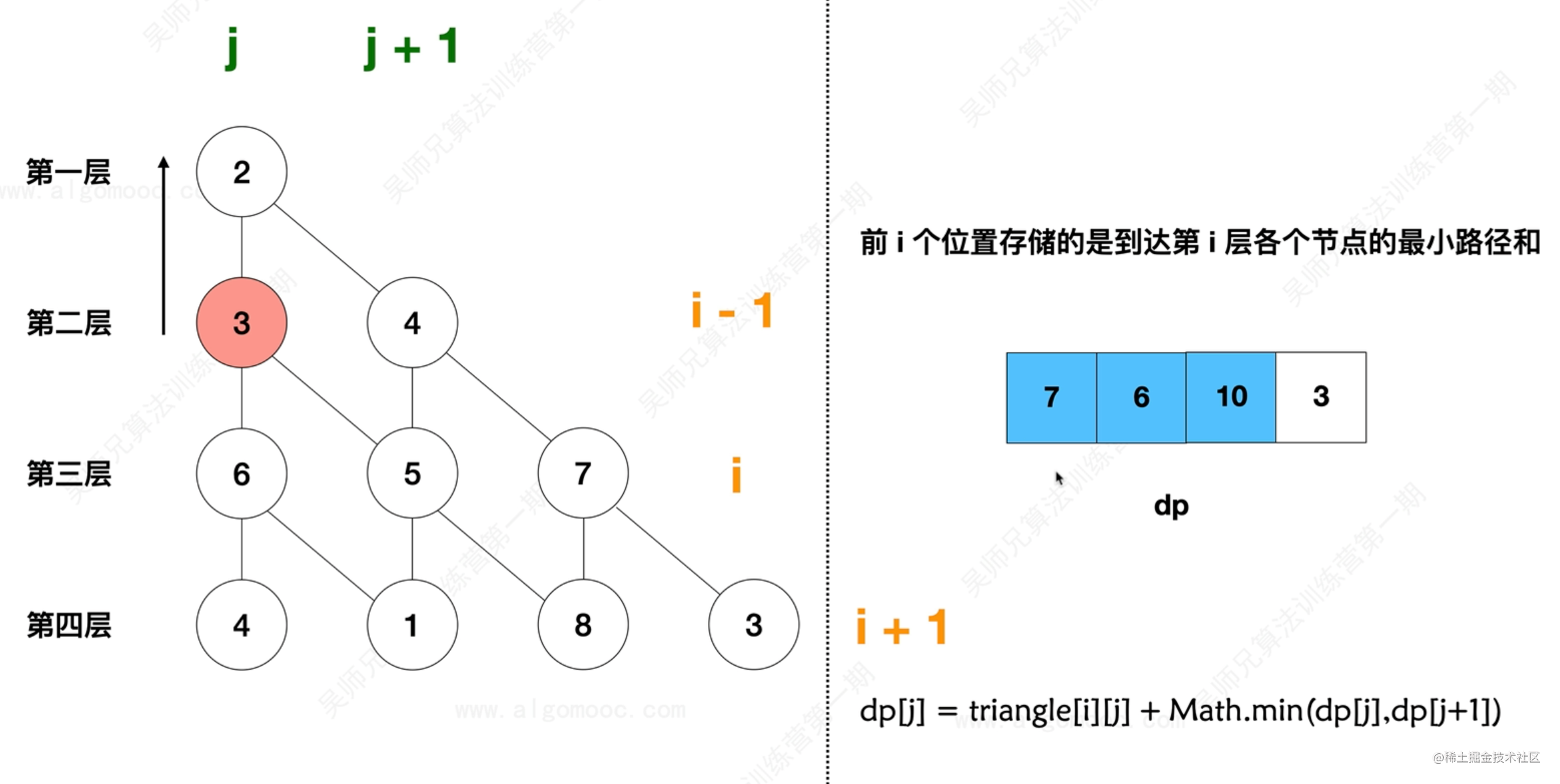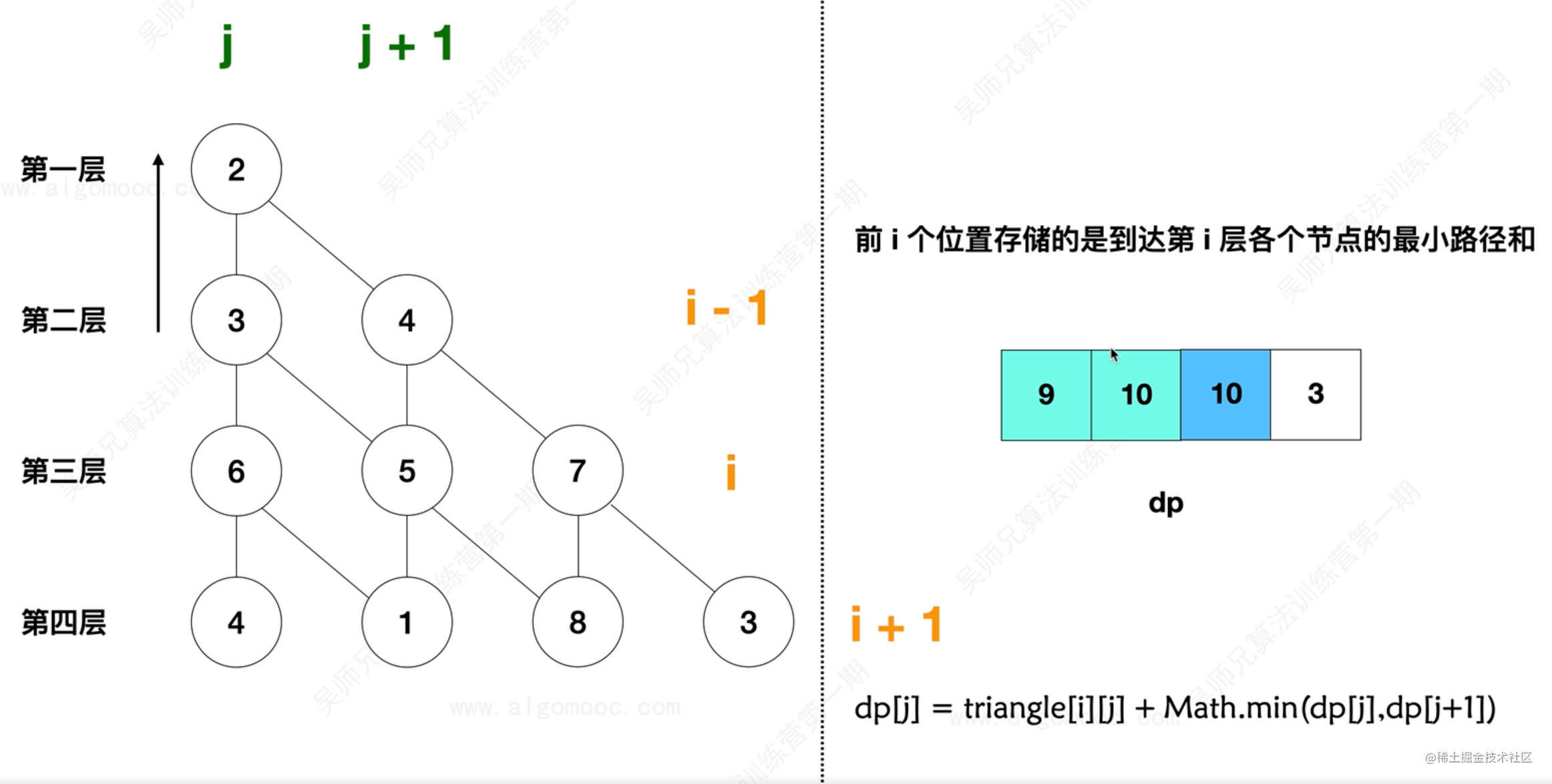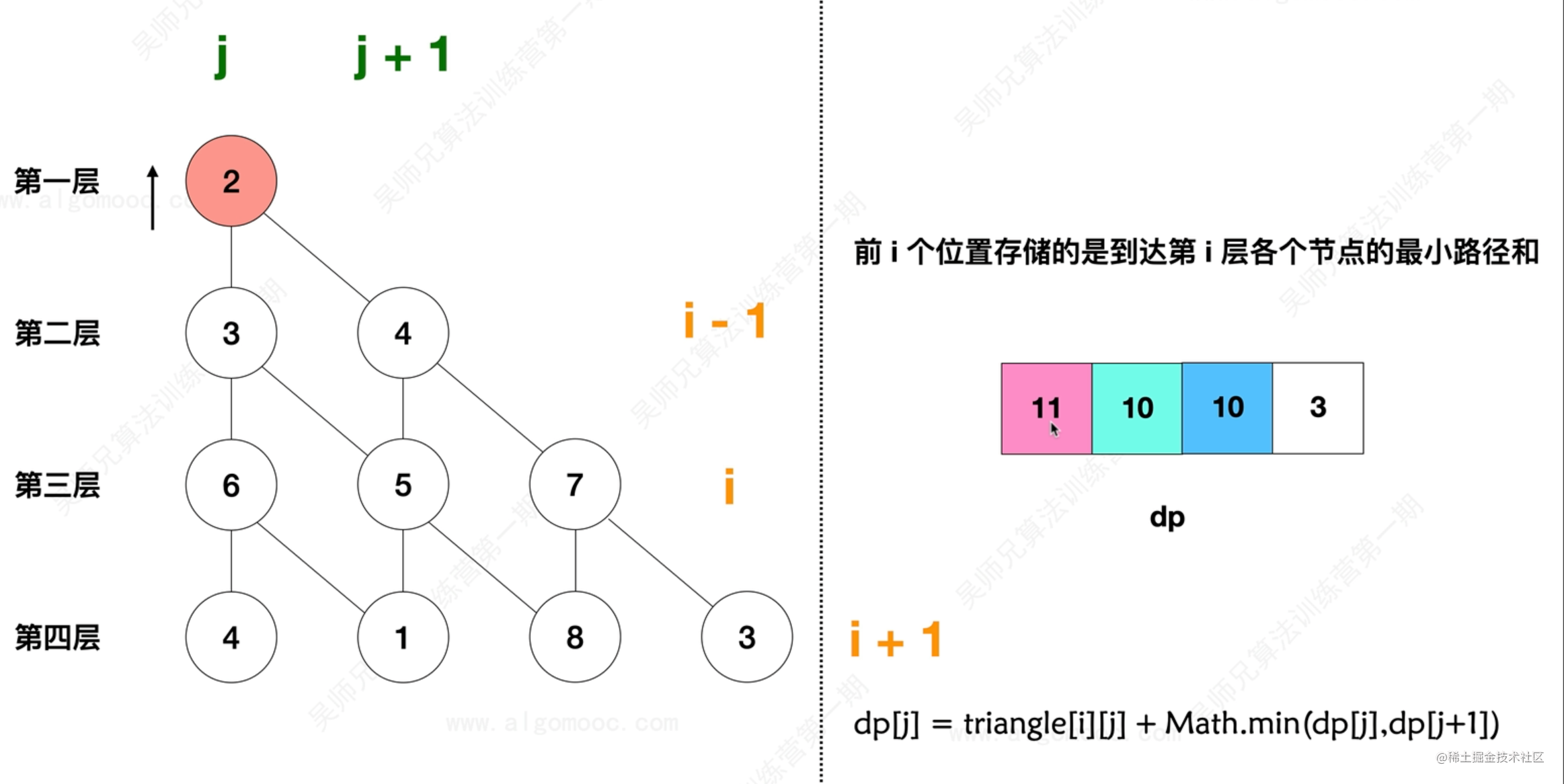``` class Solution { public int miniumTotal(List> triangle) // triangle 是個二維陣列 // 先獲取 triangle 的層數, 即一維陣列的個數 int n = triangle.size();

``````    // 設定一個一維陣列, 動態的更新每一層中當前結點對應的最短路徑
int[] dp = new int[n + 1];

// 從最後一層開始計算結點的最短路徑, 直到頂層 0層 為止
for (int i = n - 1; i >= 0; i--) {
// dp 中儲存的是前 i 個位置儲存的是到達第 i 層各個結點的最小路徑和
// 從每一層的第 0 個位置開始
for (int j = 0; j <=i ; j++) {
// dp[j] 表示第 i 層中第 j 個結點的最小路徑和
dp[j] = triangle.get(i).get(j) + Math.min(dp[j], dp[j+1]);
}
}

// 返回結果
return dp;
}
``````

} ``` - 力扣連結

#### 發文不易, 喜歡點讚的人更有好運氣👍 :), 定期更新+關注不迷路~

ps：歡迎加入筆者18年建立的研究iOS稽核及前沿技術的三千人扣群：662339934，坑位有限，備註“掘金網友”可被群管通過~